## Category: MultiCellDS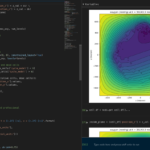The newest tool for PhysiCell provides an easy way to load your PhysiCell output data into python for analysis. This builds upon previous work on loading data into MATLAB. A post on that tool can be found at:

PhysiCell stores output data as a MultiCell Digital Snapshot (MultiCellDS) that consists of several files for each time step and is probably stored in your ./output directory. pyMCDS is a python object that is initialized with the .xml file

### What you’ll need

• python-loader, available on GitHub at
• Python 3.x, recommended distribution available at
• A number of Python packages, included in anaconda or available through pip
• NumPy
• pandas
• scipy
• Some PhysiCell data, probably in your ./output directory

### Anatomy of a MultiCell Digital Snapshot

Each time PhysiCell’s internal time tracker passes a time step where data is to be saved, it generates a number of files of various types. Each of these files will have a number at the end that indicates where it belongs in the sequence of outputs. All of the files from the first round of output will end in 00000000.* and the second round will be 00000001.* and so on. Let’s say we’re interested in a set of output from partway through the run, the 88th set of output files. The files we care about most from this set consists of:

• output00000087.xml: This file is the main organizer of the data. It contains an overview of the data stored in the MultiCellDS as well as some actual data including:
• Metadata about the time and runtime for the current time step
• Coordinates for the computational domain
• Parameters for diffusing substrates in the microenvironment
• Column labels for the cell data
• File names for the files that contain microenvironment and cell data at this time step
• output00000087_microenvironment0.mat: This is a MATLAB matrix file that contains all of the data about the microenvironment at this time step
• output00000087_cells_physicell.mat: This is a MATLAB matrix file that contains all of the tracked information about the individual cells in the model. It tells us things like the cells’ position, volume, secretion, cell cycle status, and user-defined cell parameters.

### Setup

#### Using pyMCDS

From the appropriate file in your PhysiCell directory, wherever pyMCDS.py lives, you can use the data loader in your own scripts or in an interactive session. To start you have to import the pyMCDS class

from pyMCDS import pyMCDS


Data is loaded into python from the MultiCellDS by initializing the pyMCDS object. The initialization function for pyMCDS takes one required and one optional argument.

__init__(xml_file, [output_path = '.'])
'''
xml_file : string
String containing the name of the output xml file
output_path :
String containing the path (relative or absolute) to the directory
where PhysiCell output files are stored
'''


We are interested in reading output00000087.xml that lives in ~/path/to/PhysiCell/output (don’t worry Windows paths work too). We would initialize our pyMCDS object using those names and the actual data would be stored in a member dictionary called data.

mcds = pyMCDS('output00000087.xml', '~/path/to/PhysiCell/output')
# Now our data lives in:
mcds.data


We’ve tried to keep everything organized inside of this dictionary but let’s take a look at what we actually have in here. Of course in real output, there will probably not be a chemical named my_chemical, this is simply there to illustrate how multiple chemicals are handled.The data member dictionary is a dictionary of dictionaries whose child dictionaries can be accessed through normal python dictionary syntax.

mcds.data['metadata']
mcds.data['continuum_variables']['my_chemical']


Each of these subdictionaries contains data, we will take a look at exactly what that data is and how it can be accessed in the following sections.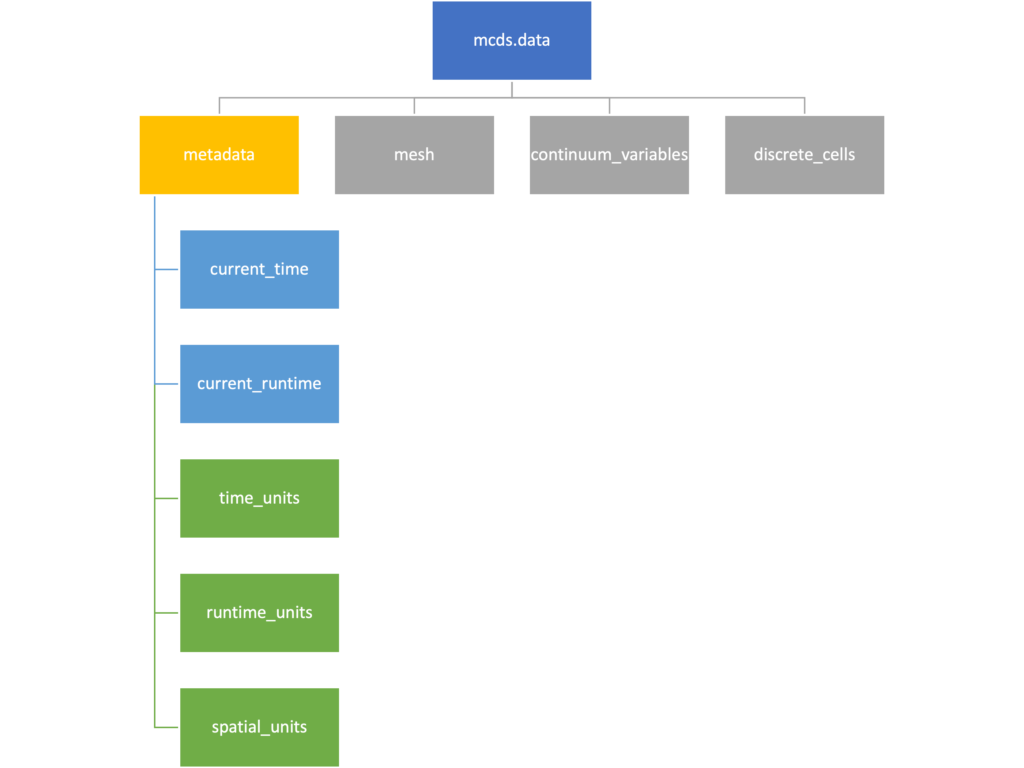The metadata dictionary contains information about the time of the simulation as well as units for both times and space. Here and in later sections blue boxes indicate scalars and green boxes indicate strings. We can access each of these things using normal dictionary syntax. We’ve also got access to a helper function get_time() for the common operation of retrieving the simulation time.

>>> mcds.data['metadata']['time_units']
'min'
>>> mcds.get_time()
5220.0


#### Mesh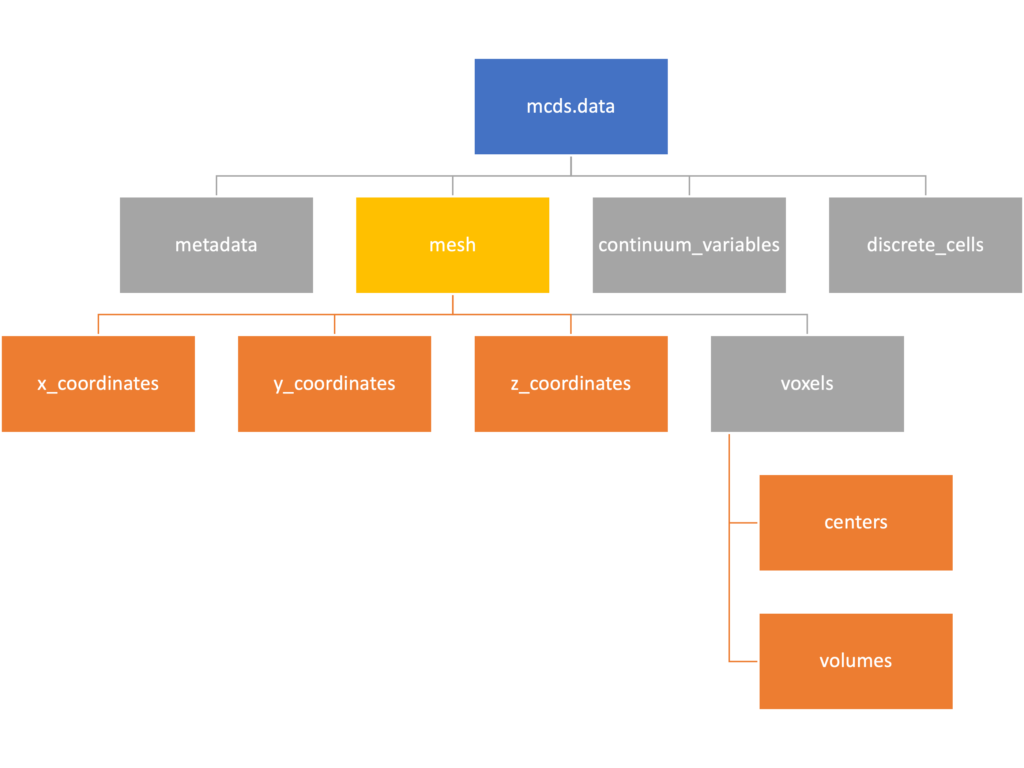The mesh dictionary has a lot more going on than the metadata dictionary. It contains three numpy arrays, indicated by orange boxes, as well as another dictionary. The three arrays contain $$x$$, $$y$$ and $$z$$ coordinates for the centers of the voxels that constiture the computational domain in a meshgrid format. This means that each of those arrays is tensors of rank three. Together they identify the coordinates of each possible point in the space.

In contrast, the arrays in the voxel dictionary are stored linearly. If we know that we care about voxel number 42, we want to use the stuff in the voxels dictionary. If we want to make a contour plot, we want to use the x_coordinates, y_coordinates, and z_coordinates arrays.

# We can extract one of the meshgrid arrays as a numpy array
>>> y_coords = mcds.data['mesh']['y_coordinates']
>>> y_coords.shape
(75, 75, 75)
>>> y_coords[0, 0, :4]
array([-740., -740., -740., -740.])

# We can also extract the array of voxel centers
>>> centers = mcds.data['mesh']['voxels']['centers']
>>> centers.shape
(3, 421875)
>>> centers[:, :4]
array([[-740., -720., -700., -680.],
[-740., -740., -740., -740.],
[-740., -740., -740., -740.]])

# We have a handy function to quickly extract the components of the full meshgrid
>>> xx, yy, zz = mcds.get_mesh()
>>> yy.shape
(75, 75, 75)
>>> yy[0, 0, :4]
array([-740., -740., -740., -740.])

# We can also use this to return the meshgrid describing an x, y plane
>>> xx, yy = mcds.get_2D_mesh()
>>> yy.shape
(75, 75)


#### Continuum variables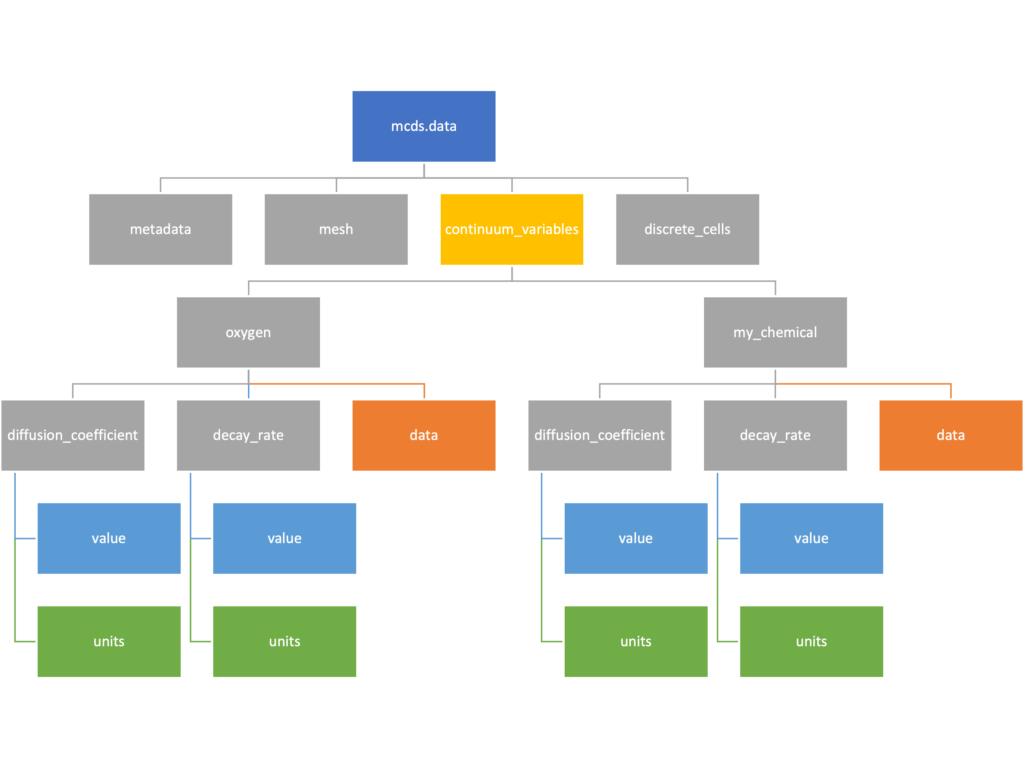The continuum_variables dictionary is the most complicated of the four. It contains subdictionaries that we access using the names of each of the chemicals in the microenvironment. In our toy example above, these are oxygen and my_chemical. If our model tracked diffusing oxygen, VEGF, and glucose, then the continuum_variables dictionary would contain a subdirectory for each of them.

For a particular chemical species in the microenvironment we have two more dictionaries called decay_rate and diffusion_coefficient, and a numpy array called data. The diffusion and decay dictionaries each complete the value stored as a scalar and the unit stored as a string. The numpy array contains the concentrations of the chemical in each voxel at this time and is the same shape as the meshgrids of the computational domain stored in the .data[‘mesh’] arrays.

# we need to know the names of the substrates to work with
# this data. We have a function to help us find them.
>>> mcds.get_substrate_names()
['oxygen', 'my_chemical']

# The diffusable chemical dictionaries are messy
# if we need to do a lot with them it might be easier
# to put them into their own instance
>>> oxy_dict = mcds.data['continuum_variables']['oxygen']
>>> oxy_dict['decay_rate']
{'value': 0.1, 'units': '1/min'}

# What we care about most is probably the numpy
# array of concentrations
>>> oxy_conc = oxy_dict['data']
>>> oxy_conc.shape
(75, 75, 75)

# Alternatively, we can get the same array with a function
>>> oxy_conc2 = mcds.get_concentrations('oxygen')
>>> oxy_conc2.shape
(75, 75, 75)

# We can also get the concentrations on a plane using the
# same function and supplying a z value to "slice through"
# note that right now the z_value must be an exact match
# for a plane of voxel centers, in the future we may add
# interpolation.
>>> oxy_plane = mcds.get_concentrations('oxygen', z_value=100.0)
>>> oxy_plane.shape
(75, 75)

# we can also find the concentration in a single voxel using the
# position of a point within that voxel. This will give us an
# array of all concentrations at that point.
>>> mcds.get_concentrations_at(x=0., y=550., z=0.)
array([17.94514446,  0.99113448])


#### Discrete Cells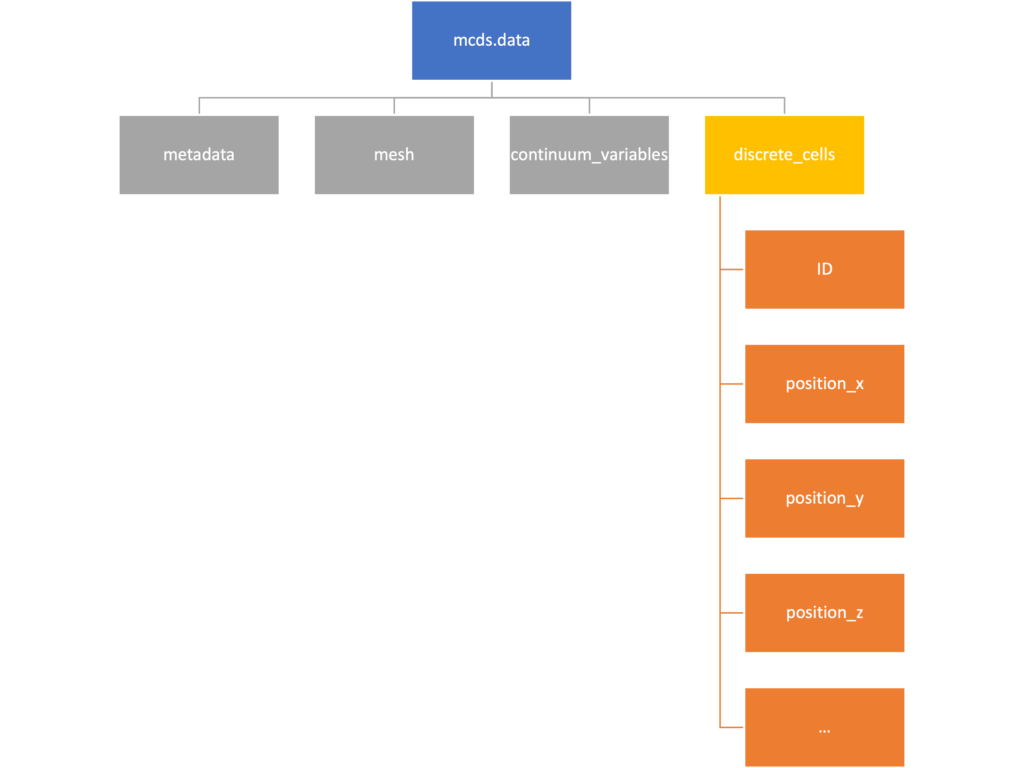The discrete cells dictionary is relatively straightforward. It contains a number of numpy arrays that contain information regarding individual cells.  These are all 1-dimensional arrays and each corresponds to one of the variables specified in the output*.xml file. With the default settings, these are:

• ID: unique integer that will identify the cell throughout its lifetime in the simulation
• position(_x, _y, _z): floating point positions for the cell in $$x$$, $$y$$, and $$z$$ directions
• total_volume: total volume of the cell
• cell_type: integer label for the cell as used in PhysiCell
• cycle_model: integer label for the cell cycle model as used in PhysiCell
• current_phase: integer specification for which phase of the cycle model the cell is currently in
• elapsed_time_in_phase: time that cell has been in current phase of cell cycle model
• nuclear_volume: volume of cell nucleus
• cytoplasmic_volume: volume of cell cytoplasm
• fluid_fraction: proportion of the volume due to fliud
• calcified_fraction: proportion of volume consisting of calcified material
• orientation(_x, _y, _z): direction in which cell is pointing
• polarity:
• migration_speed: current speed of cell
• motility_vector(_x, _y, _z): current direction of movement of cell
• migration_bias: coefficient for stochastic movement (higher is “more deterministic”)
• motility_bias_direction(_x, _y, _z): direction of movement bias
• persistence_time: time in-between direction changes for cell
• motility_reserved:
# Extracting single variables is just like before
>>> cell_ids = mcds.data['discrete_cells']['ID']
>>> cell_ids.shape
(18595,)
>>> cell_ids[:4]
array([0., 1., 2., 3.])

# If we're clever we can extract 2D arrays
>>> cell_vec = np.zeros((cell_ids.shape, 3))
>>> vec_list = ['position_x', 'position_y', 'position_z']
>>> for i, lab in enumerate(vec_list):
...     cell_vec[:, i] = mcds.data['discrete_cells'][lab]
...
array([[ -69.72657128,  -39.02046405, -233.63178904],
[ -69.84507464,  -22.71693265, -233.59277388],
[ -69.84891462,   -6.04070516, -233.61816711],
[ -69.845265  ,   10.80035554, -233.61667313]])

# We can get the list of all of the variables stored in this dictionary
>>> mcds.get_cell_variables()
['ID',
'position_x',
'position_y',
'position_z',
'total_volume',
'cell_type',
'cycle_model',
'current_phase',
'elapsed_time_in_phase',
'nuclear_volume',
'cytoplasmic_volume',
'fluid_fraction',
'calcified_fraction',
'orientation_x',
'orientation_y',
'orientation_z',
'polarity',
'migration_speed',
'motility_vector_x',
'motility_vector_y',
'motility_vector_z',
'migration_bias',
'motility_bias_direction_x',
'motility_bias_direction_y',
'motility_bias_direction_z',
'persistence_time',
'motility_reserved',
'oncoprotein',
'elastic_coefficient',
'kill_rate',
'attachment_rate']
# We can also get all of the cell data as a pandas DataFrame
>>> cell_df = mcds.get_cell_df()
ID     position_x   position_y    position_z total_volume cell_type cycle_model ...
0.0   - 69.726571  - 39.020464  - 233.631789       2494.0       0.0         5.0 ...
1.0   - 69.845075  - 22.716933  - 233.592774       2494.0       0.0         5.0 ...
2.0   - 69.848915  - 6.040705   - 233.618167       2494.0       0.0         5.0 ...
3.0   - 69.845265    10.800356  - 233.616673       2494.0       0.0         5.0 ...
4.0   - 69.828161    27.324530  - 233.631579       2494.0       0.0         5.0 ...

# if we want to we can also get just the subset of cells that
# are in a specific voxel
>>> vox_df = mcds.get_cell_df_at(x=0.0, y=550.0, z=0.0)
>>> vox_df.iloc[:, :5]
ID  position_x  position_y  position_z  total_volume
26718  228761.0    6.623617  536.709341   -1.282934   2454.814507
52736  270274.0   -7.990034  538.184921    9.648955   1523.386488


### Examples

These examples will not be made using our toy dataset described above but will instead be made using a single timepoint dataset that can be found at:

#### Substrate contour plot

One of the big advantages of working with PhysiCell data in python is that we have access to its plotting tools. For the sake of example let’s plot the partial pressure of oxygen throughout the computational domain along the $$z = 0$$ plane. Once we’ve loaded our data by initializing a pyMCDS object, we can work entirely within python to produce the plot.

from pyMCDS import pyMCDS
import numpy as np
import matplotlib.pyplot as plt

mcds = pyMCDS('output00003696.xml', '../output')

# Set our z plane and get our substrate values along it
z_val = 0.00
plane_oxy = mcds.get_concentrations('oxygen', z_slice=z_val)

# Get the 2D mesh for contour plotting
xx, yy = mcds.get_mesh()

# We want to be able to control the number of contour levels so we
# need to do a little set up
num_levels = 21
min_conc = plane_oxy.min()
max_conc = plane_oxy.max()
my_levels = np.linspace(min_conc, max_conc, num_levels)

# set up the figure area and add data layers
fig, ax = plt.subplot()
cs = ax.contourf(xx, yy, plane_oxy, levels=my_levels)
ax.contour(xx, yy, plane_oxy, color='black', levels = my_levels,
linewidths=0.5)

# Now we need to add our color bar
cbar1 = fig.colorbar(cs, shrink=0.75)
cbar1.set_label('mmHg')

# Let's put the time in to make these look nice
ax.set_aspect('equal')
ax.set_xlabel('x (micron)')
ax.set_ylabel('y (micron)')
ax.set_title('oxygen (mmHg) at t = {:.1f} {:s}, z = {:.2f} {:s}'.format(
mcds.get_time(),
z_val,

plt.show()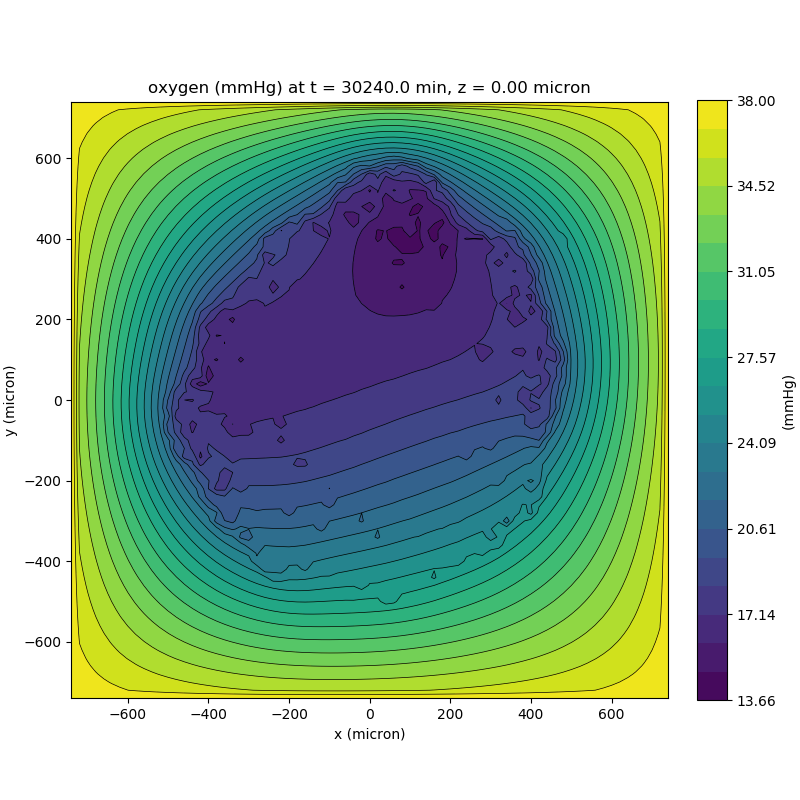We can also use pandas to do fairly complex selections of cells to add to our plots. Below we use pandas and the previous plot to add a cells layer.

from pyMCDS import pyMCDS
import numpy as np
import matplotlib.pyplot as plt

mcds = pyMCDS('output00003696.xml', '../output')

# Set our z plane and get our substrate values along it
z_val = 0.00
plane_oxy = mcds.get_concentrations('oxygen', z_slice=z_val)

# Get the 2D mesh for contour plotting
xx, yy = mcds.get_mesh()

# We want to be able to control the number of contour levels so we
# need to do a little set up
num_levels = 21
min_conc = plane_oxy.min()
max_conc = plane_oxy.max()
my_levels = np.linspace(min_conc, max_conc, num_levels)

# get our cells data and figure out which cells are in the plane
cell_df = mcds.get_cell_df()
ds = mcds.get_mesh_spacing()
inside_plane = (cell_df['position_z'] < z_val + ds) \ & (cell_df['position_z'] > z_val - ds)
plane_cells = cell_df[inside_plane]

# We're going to plot two types of cells and we want it to look nice
colors = ['black', 'grey']
sizes = [20, 8]

# set up the figure area and add microenvironment layer
fig, ax = plt.subplot()
cs = ax.contourf(xx, yy, plane_oxy, levels=my_levels)

# get our cells of interest
# alive_cells = plane_cells[plane_cells['cycle_model'] < 6]
# dead_cells = plane_cells[plane_cells['cycle_model'] > 6]
# -- for newer versions of PhysiCell
alive_cells = plane_cells[plane_cells['cycle_model'] < 100]

# plot the cell layer
for i, plot_cells in enumerate((alive_cells, dead_cells)):
ax.scatter(plot_cells['position_x'].values,
plot_cells['position_y'].values,
facecolor='none',
edgecolors=colors[i],
alpha=0.6,
s=sizes[i],
label=labels[i])

# Now we need to add our color bar
cbar1 = fig.colorbar(cs, shrink=0.75)
cbar1.set_label('mmHg')

# Let's put the time in to make these look nice
ax.set_aspect('equal')
ax.set_xlabel('x (micron)')
ax.set_ylabel('y (micron)')
ax.set_title('oxygen (mmHg) at t = {:.1f} {:s}, z = {:.2f} {:s}'.format(
mcds.get_time(),
z_val,
ax.legend(loc='upper right')

plt.show()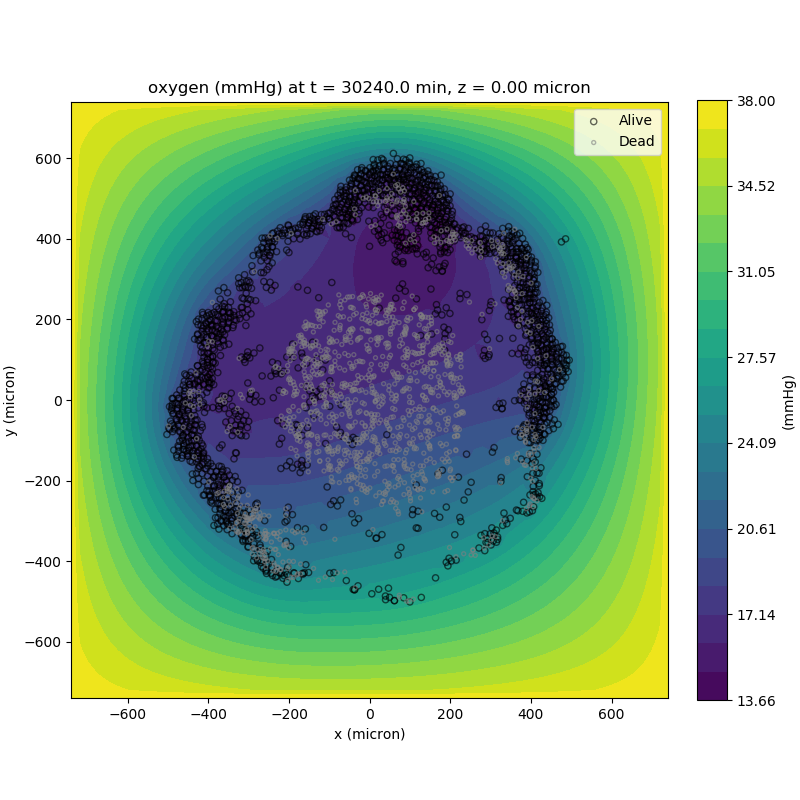#### Future Direction

The first extension of this project will be timeseries functionality. This will provide similar data loading functionality but for a time series of MultiCell Digital Snapshots instead of simply one point in time.

## PhysiCell Tools : PhysiCell-povwriter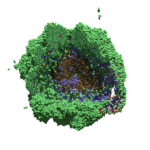As PhysiCell matures, we are starting to turn our attention to better training materials and an ecosystem of open source PhysiCell tools. PhysiCell-povwriter is is designed to help transform your 3-D simulation results into 3-D visualizations like this one:

PhysiCell-povwriter transforms simulation snapshots into 3-D scenes that can be rendered into still images using POV-ray: an open source software package that uses raytracing to mimic the path of light from a source of illumination to a single viewpoint (a camera or an eye). The result is a beautifully rendered scene (at any resolution you choose) with very nice shading and lighting.

If you repeat this on many simulation snapshots, you can create an animation of your work.

### What you’ll need

This workflow is entirely based on open source software:

• 3-D simulation data (likely stored in ./output from your project)
• PhysiCell-povwriter, available on GitHub at
• POV-ray, available at
• ImageMagick (optional, for image file conversions)
• mencoder (optional, for making compressed movies)

### Setup

#### Building PhysiCell-povwriter

After you clone PhysiCell-povwriter or download its source from a release, you’ll need to compile it. In the project’s root directory, compile the project by:

make


(If you need to set up a C++ PhysiCell development environment, click here for OSX or here for Windows.)

Next, copy povwriter (povwriter.exe in Windows) to either the root directory of your PhysiCell project, or somewhere in your path. Copy ./config/povwriter-settings.xml to the ./config directory of your PhysiCell project.

#### Editing resolutions in POV-ray

PhysiCell-povwriter is intended for creating “square” images, but POV-ray does not have any pre-created square rendering resolutions out-of-the-box. However, this is straightforward to fix.

1. Open POV-Ray
2. Go to the “tools” menu and select “edit resolution INI file”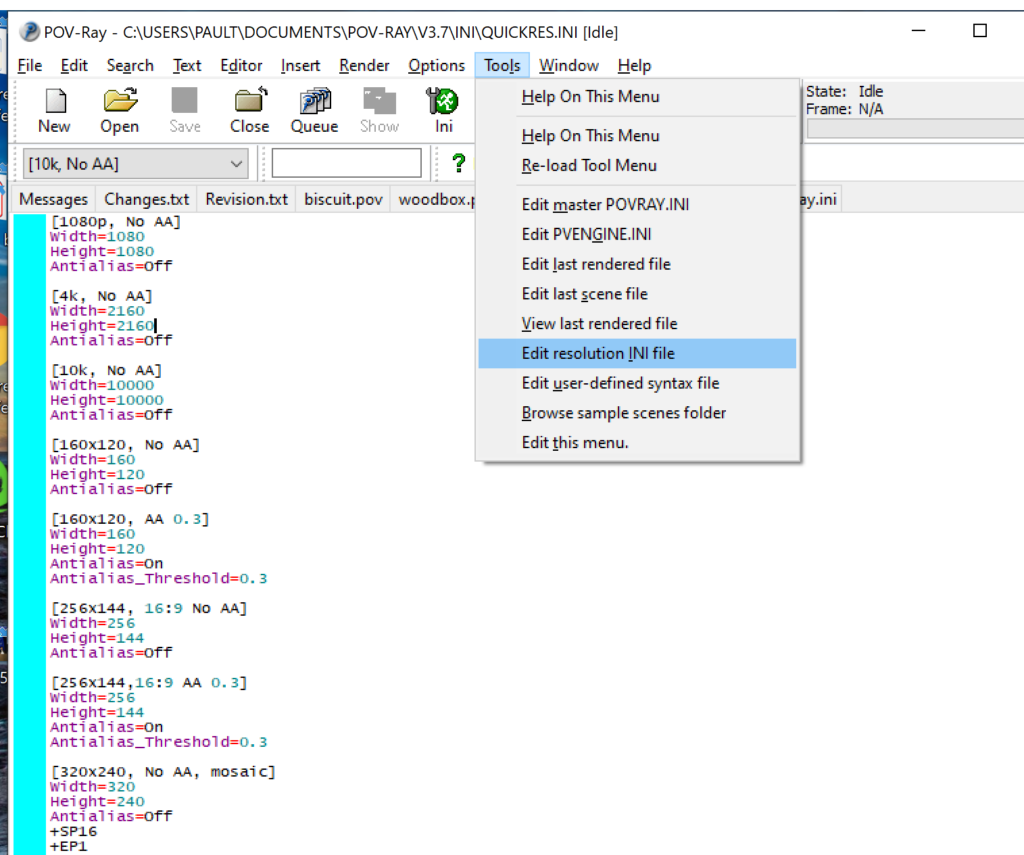3. At the top of the INI file (which opens for editing in POV-ray), make a new profile:
[1080x1080, AA]
Width=480
Height=480
Antialias=On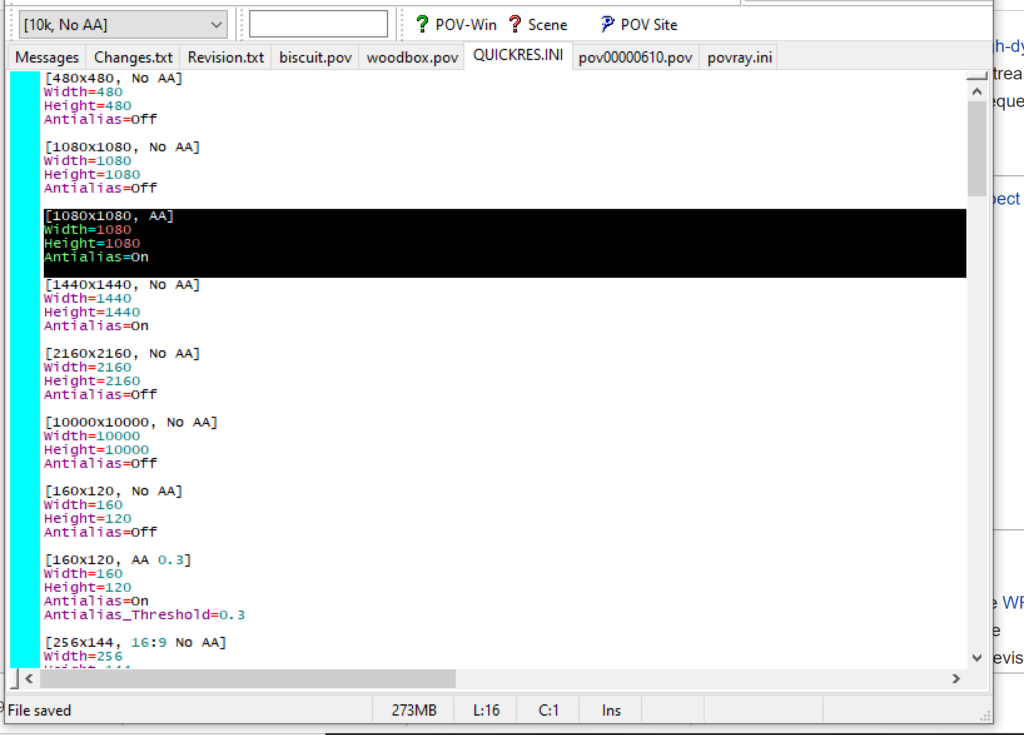4. Make similar profiles (with unique names) to suit your preferences. I suggest one at 480×480 (as a fast preview), another at 2160×2160, and another at 5000×5000 (because they will be absurdly high resolution). For example:
[2160x2160 no AA]
Width=2160
Height=2160
Antialias=Off


You can optionally make more profiles with antialiasing on (which provides some smoothing for areas of high detail), but you’re probably better off just rendering without antialiasing at higher resolutions and the scaling the image down as needed. Also, rendering without antialiasing will be faster.

5. Once done making profiles, save and exit POV-Ray.
6. The next time you open POV-Ray, your new resolution profiles will be available in the lefthand dropdown box.

### Configuring PhysiCell-povwriter

Once you have copied povwriter-settings.xml to your project’s config file, open it in a text editor. Below, we’ll show the different settings.

#### Camera settings

<camera>
<distance_from_origin units="micron">1500</distance_from_origin>
<xy_angle>3.92699081699</xy_angle> <!-- 5*pi/4 -->
<yz_angle>1.0471975512</yz_angle> <!-- pi/3 -->
</camera>


For simplicity, PhysiCell-POVray (currently) always aims the camera towards the origin (0,0,0), with “up” towards the positive z-axis. distance_from_origin sets how far the camera is placed from the origin. xy_angle sets the angle $$\theta$$ from the positive x-axis in the xy-plane. yz_angle sets the angle $$\phi$$ from the positive z-axis in the yz-plane. Both angles are in radians.

#### Options

<options>
<use_standard_colors>true</use_standard_colors>
<nuclear_offset units="micron">0.1</nuclear_offset>
<cell_bound units="micron">750</cell_bound>
</options>


use_standard_colors (if set to true) uses a built-in “paint-by-numbers” color scheme, where each cell type (identified with an integer) gets XML-defined colors for live, apoptotic, and dead cells. More on this below. If use_standard_colors is set to false, then PhysiCell-povwriter uses the my_pigment_and_finish_function in ./custom_modules/povwriter.cpp to color cells.

The nuclear_offset is a small additional height given to nuclei when cropping to avoid visual artifacts when rendering (which can cause some “tearing” or “bleeding” between the rendered nucleus and cytoplasm). cell_bound is used for leaving some cells out of bound: any cell with |x|, |y|, or |z| exceeding cell_bound will not be rendered. threads is used for parallelizing on multicore processors; note that it only speeds up povwriter if you are converting multiple PhysiCell outputs to povray files.

#### Save

<save> <!-- done -->
<folder>output</folder> <!-- use . for root -->
<filebase>output</filebase>
<time_index>3696</time_index>
</save>


Use folder to tell PhysiCell-povwriter where the data files are stored. Use filebase to tell how the outputs are named. Typically, they have the form output########_cells_physicell.mat; in this case, the filebase is output. Lastly, use time_index to set the output number. For example if your file is output00000182_cells_physicell.mat, then filebase = output and time_index = 182.

Below, we’ll see how to specify ranges of indices at the command line, which would supersede the time_index given here in the XML.

#### Clipping planes

PhysiCell-povwriter uses clipping planes to help create cutaway views of the simulations. By default, 3 clipping planes are used to cut out an octant of the viewing area.

Recall that a plane can be defined by its normal vector and a point p on the plane. With these, the plane can be defined as all points satisfying

$\left( \vec{x} -\vec{p} \right) \cdot \vec{n} = 0$

These are then written out as a plane equation

$a x + by + cz + d = 0,$

where

$(a,b,c) = \vec{n} \hspace{.5in} \textrm{ and } \hspace{0.5in} d = \: – \vec{n} \cdot \vec{p}.$

As of Version 1.0.0, we are having some difficulties with clipping planes that do not pass through the origin (0,0,0), for which $$d = 0$$.

In the config file, these planes are written as $$(a,b,c,d)$$:

<clipping_planes> <!-- done -->
<clipping_plane>0,-1,0,0</clipping_plane>
<clipping_plane>-1,0,0,0</clipping_plane>
<clipping_plane>0,0,1,0</clipping_plane>
</clipping_planes>


Note that cells “behind” the plane (where $$( \vec{x} – \vec{p} ) \cdot \vec{n} \le 0$$) are rendered, and cells in “front” of the plane (where $$(\vec{x}-\vec{p}) \cdot \vec{n} > 0$$) are not rendered. Cells that intersect the plane are partially rendered (using constructive geometry via union and intersection commands in POV-ray).

#### Cell color definitions

Within <cell_color_definitions>, you’ll find multiple <cell_colors> blocks, each of which defines the live, dead, and necrotic colors for a specific cell type (with the type ID indicated in the attribute). These colors are only applied if use_standard_colors is set to true in options. See above.

The live colors are given as two rgb (red,green,blue) colors for the cytoplasm and nucleus of live cells. Each element of this triple can range from 0 to 1, and not from 0 to 255 as in many raw image formats. Next, finish specifies ambient (how much highly-scattered background ambient light illuminates the cell), diffuse (how well light rays can illuminate the surface), and specular (how much of a shiny reflective splotch the cell gets).

This is repeated to give the apoptotic and necrotic colors for the cell type.

<cell_colors type="0">
<live>
<cytoplasm>.25,1,.25</cytoplasm> <!-- red,green,blue -->
<nuclear>0.03,0.125</nuclear>
<finish>0.05,1,0.1</finish> <!-- ambient,diffuse,specular -->
</live>
<apoptotic>
<cytoplasm>1,0,0</cytoplasm> <!-- red,green,blue -->
<nuclear>0.125,0,0</nuclear>
<finish>0.05,1,0.1</finish> <!-- ambient,diffuse,specular -->
</apoptotic>
<necrotic>
<cytoplasm>1,0.5412,0.1490</cytoplasm> <!-- red,green,blue -->
<nuclear>0.125,0.06765,0.018625</nuclear>
<finish>0.01,0.5,0.1</finish> <!-- ambient,diffuse,specular -->
</necrotic>
</cell_colors>


Use multiple cell_colors blocks (each with type corresponding to the integer cell type) to define the colors of multiple cell types.

### Using PhysiCell-povwriter

#### Use by the XML configuration file alone

The simplest syntax:

physicell$./povwriter  (Windows users: povwriter or povwriter.exe) will process ./config/povwriter-settings.xml and convert the single indicated PhysiCell snapshot to a .pov file. If you run POV-writer with the default configuration file in the povwriter structure (with the supplied sample data), it will render time index 3696 from the immunotherapy example in our 2018 PhysiCell Method Paper: physicell$ ./povwriter

povwriter version 1.0.0
================================================================================

Copyright (c) Paul Macklin 2019, on behalf of the PhysiCell project

Usage:
================================================================================
povwriter : run povwriter with config file ./config/settings.xml

povwriter FILENAME.xml : run povwriter with config file FILENAME.xml

povwriter x:y:z : run povwriter on data in FOLDER with indices from x
to y in incremenets of z

Example: ./povwriter 0:2:10 processes files:
./FOLDER/FILEBASE00000000_physicell_cells.mat
./FOLDER/FILEBASE00000002_physicell_cells.mat
...
./FOLDER/FILEBASE00000010_physicell_cells.mat
(See the config file to set FOLDER and FILEBASE)

povwriter x1,...,xn : run povwriter on data in FOLDER with indices x1,...,xn

Example: ./povwriter 1,3,17 processes files:
./FOLDER/FILEBASE00000001_physicell_cells.mat
./FOLDER/FILEBASE00000003_physicell_cells.mat
./FOLDER/FILEBASE00000017_physicell_cells.mat
(Note that there are no spaces.)
(See the config file to set FOLDER and FILEBASE)

Tutorial & documentation at http://MathCancer.org/blog/povwriter
================================================================================

Using config file ./config/povwriter-settings.xml ...
Using standard coloring function ...
Found 3 clipping planes ...
Found 2 cell color definitions ...
Processing file ./output/output00003696_cells_physicell.mat...
Matrix size: 32 x 66978
Creating file pov00003696.pov for output ...
Writing 66978 cells ...
done!

Done processing all 1 files!


The result is a single POV-ray file (pov00003696.pov) in the root directory.

Now, open that file in POV-ray (double-click the file if you are in Windows), choose one of your resolutions in your lefthand dropdown (I’ll choose 2160×2160 no antialiasing), and click the green “run” button.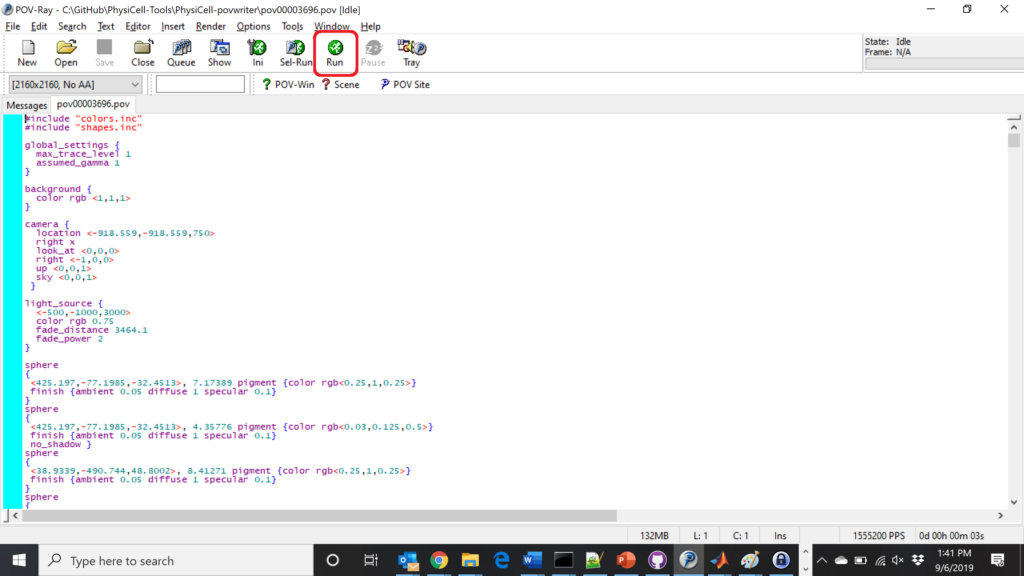You can watch the image as it renders. The result should be a PNG file (named pov00003696.png) that looks like this:

#### Using command-line options to process multiple times (option #1)

Now, suppose we have more outputs to process. We still state most of the options in the XML file as above, but now we also supply a command-line argument in the form of start:interval:end. If you’re still in the povwriter project, note that we have some more sample data there. Let’s grab and process it:

physicell$cd output physicell$ unzip more_samples.zip
Archive: more_samples.zip
inflating: output00000000_cells_physicell.mat
inflating: output00000001_cells_physicell.mat
inflating: output00000250_cells_physicell.mat
inflating: output00000300_cells_physicell.mat
inflating: output00000500_cells_physicell.mat
inflating: output00000750_cells_physicell.mat
inflating: output00001000_cells_physicell.mat
inflating: output00001250_cells_physicell.mat
inflating: output00001500_cells_physicell.mat
inflating: output00001750_cells_physicell.mat
inflating: output00002000_cells_physicell.mat
inflating: output00002250_cells_physicell.mat
inflating: output00002500_cells_physicell.mat
inflating: output00002750_cells_physicell.mat
inflating: output00003000_cells_physicell.mat
inflating: output00003250_cells_physicell.mat
inflating: output00003500_cells_physicell.mat
inflating: output00003696_cells_physicell.mat

physicell$ls citation and license.txt more_samples.zip output00000000_cells_physicell.mat output00000001_cells_physicell.mat output00000250_cells_physicell.mat output00000300_cells_physicell.mat output00000500_cells_physicell.mat output00000750_cells_physicell.mat output00001000_cells_physicell.mat output00001250_cells_physicell.mat output00001500_cells_physicell.mat output00001750_cells_physicell.mat output00002000_cells_physicell.mat output00002250_cells_physicell.mat output00002500_cells_physicell.mat output00002750_cells_physicell.mat output00003000_cells_physicell.mat output00003250_cells_physicell.mat output00003500_cells_physicell.mat output00003696.xml output00003696_cells_physicell.mat  Let’s go back to the parent directory and run povwriter: physicell$ ./povwriter 0:250:3500

povwriter version 1.0.0
================================================================================

Copyright (c) Paul Macklin 2019, on behalf of the PhysiCell project

Usage:
================================================================================
povwriter : run povwriter with config file ./config/settings.xml

povwriter FILENAME.xml : run povwriter with config file FILENAME.xml

povwriter x:y:z : run povwriter on data in FOLDER with indices from x
to y in incremenets of z

Example: ./povwriter 0:2:10 processes files:
./FOLDER/FILEBASE00000000_physicell_cells.mat
./FOLDER/FILEBASE00000002_physicell_cells.mat
...
./FOLDER/FILEBASE00000010_physicell_cells.mat
(See the config file to set FOLDER and FILEBASE)

povwriter x1,...,xn : run povwriter on data in FOLDER with indices x1,...,xn

Example: ./povwriter 1,3,17 processes files:
./FOLDER/FILEBASE00000001_physicell_cells.mat
./FOLDER/FILEBASE00000003_physicell_cells.mat
./FOLDER/FILEBASE00000017_physicell_cells.mat
(Note that there are no spaces.)
(See the config file to set FOLDER and FILEBASE)

Tutorial & documentation at http://MathCancer.org/blog/povwriter
================================================================================

Using config file ./config/povwriter-settings.xml ...
Using standard coloring function ...
Found 3 clipping planes ...
Found 2 cell color definitions ...
Matrix size: 32 x 18317
Processing file ./output/output00000000_cells_physicell.mat...
Creating file pov00000000.pov for output ...
Writing 18317 cells ...
Processing file ./output/output00002000_cells_physicell.mat...
Matrix size: 32 x 33551
Creating file pov00002000.pov for output ...
Writing 33551 cells ...
Processing file ./output/output00002500_cells_physicell.mat...
Matrix size: 32 x 43440
Creating file pov00002500.pov for output ...
Writing 43440 cells ...
Processing file ./output/output00001500_cells_physicell.mat...
Matrix size: 32 x 40267
Creating file pov00001500.pov for output ...
Writing 40267 cells ...
Processing file ./output/output00003000_cells_physicell.mat...
Matrix size: 32 x 56659
Creating file pov00003000.pov for output ...
Writing 56659 cells ...
Processing file ./output/output00001000_cells_physicell.mat...
Matrix size: 32 x 74057
Creating file pov00001000.pov for output ...
Writing 74057 cells ...
Processing file ./output/output00003500_cells_physicell.mat...
Matrix size: 32 x 66791
Creating file pov00003500.pov for output ...
Writing 66791 cells ...
Processing file ./output/output00000500_cells_physicell.mat...
Matrix size: 32 x 114316
Creating file pov00000500.pov for output ...
Writing 114316 cells ...
done!

Processing file ./output/output00000250_cells_physicell.mat...
Matrix size: 32 x 75352
Creating file pov00000250.pov for output ...
Writing 75352 cells ...
done!

Processing file ./output/output00002250_cells_physicell.mat...
Matrix size: 32 x 37959
Creating file pov00002250.pov for output ...
Writing 37959 cells ...
done!

Processing file ./output/output00001750_cells_physicell.mat...
Matrix size: 32 x 32358
Creating file pov00001750.pov for output ...
Writing 32358 cells ...
done!

Processing file ./output/output00002750_cells_physicell.mat...
Matrix size: 32 x 49658
Creating file pov00002750.pov for output ...
Writing 49658 cells ...
done!

Processing file ./output/output00003250_cells_physicell.mat...
Matrix size: 32 x 63546
Creating file pov00003250.pov for output ...
Writing 63546 cells ...
done!

done!

done!

done!

Processing file ./output/output00001250_cells_physicell.mat...
Matrix size: 32 x 54771
Creating file pov00001250.pov for output ...
Writing 54771 cells ...
done!

done!

done!

done!

Processing file ./output/output00000750_cells_physicell.mat...
Matrix size: 32 x 97642
Creating file pov00000750.pov for output ...
Writing 97642 cells ...
done!

done!

Done processing all 15 files!


Notice that the output appears a bit out of order. This is normal: povwriter is using 8 threads to process 8 files at the same time, and sending some output to the single screen. Since this is all happening simultaneously, it’s a bit jumbled (and non-sequential). Don’t panic. You should now have created pov00000000.povpov00000250.pov, … , pov00003500.pov.

Now, go into POV-ray, and choose “queue.” Click “Add File” and select all 15 .pov files you just created: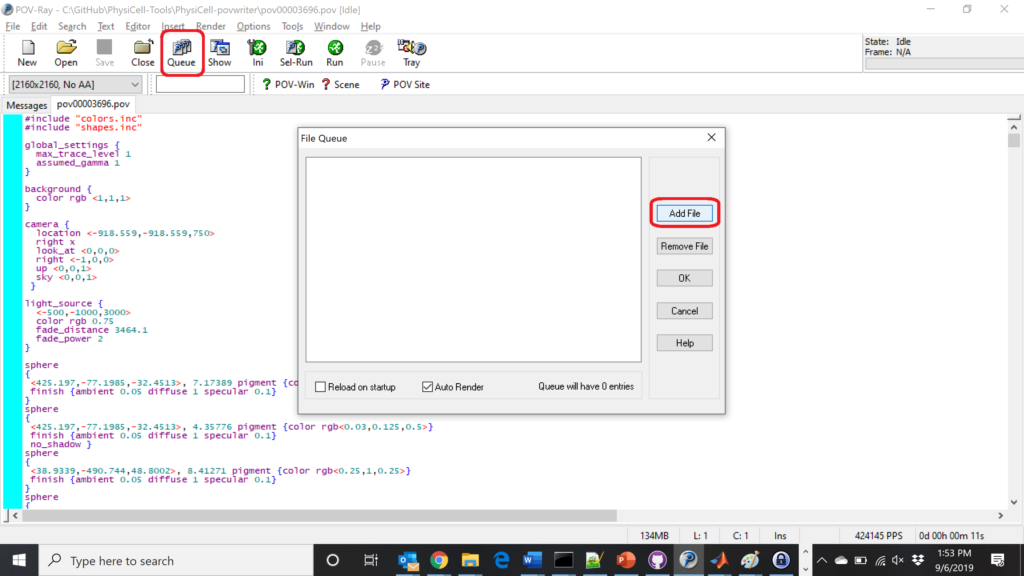Hit “OK” to let it render all the povray files to create PNG files (pov00000000.png, … , pov00003500.png).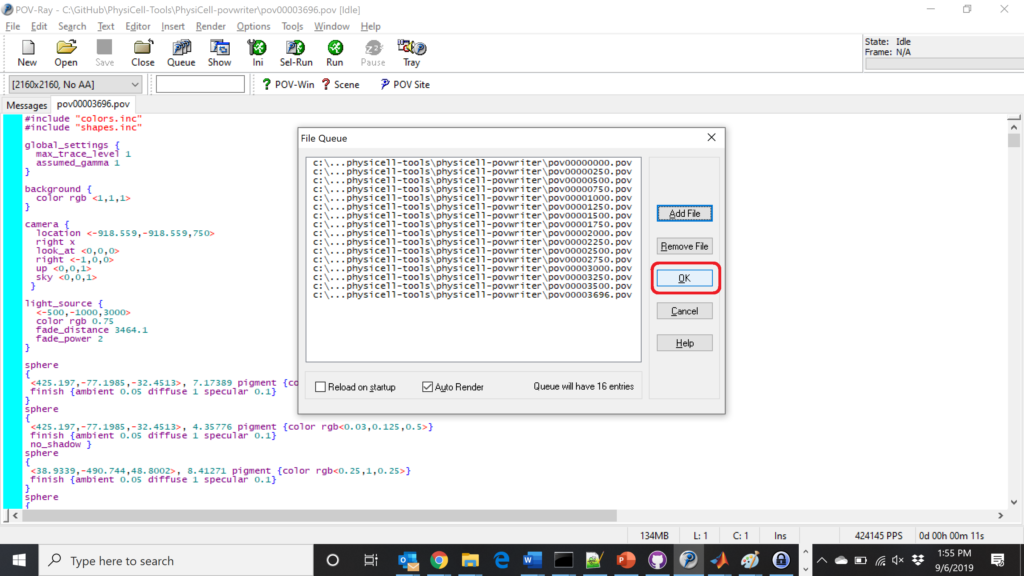#### Using command-line options to process multiple times (option #2)

You can also give a list of indices. Here’s how we render time indices 250, 1000, and 2250:



#### Creating an animated GIF with ImageMagick

Suppose you want to create an animated GIF based on your images. I suggest first converting to JPG (see above) and then using ImageMagick again. Here, I’m adding a 20 ms delay between frames:

physicell$magick convert -delay 20 *.jpg out.gif  Here’s the result: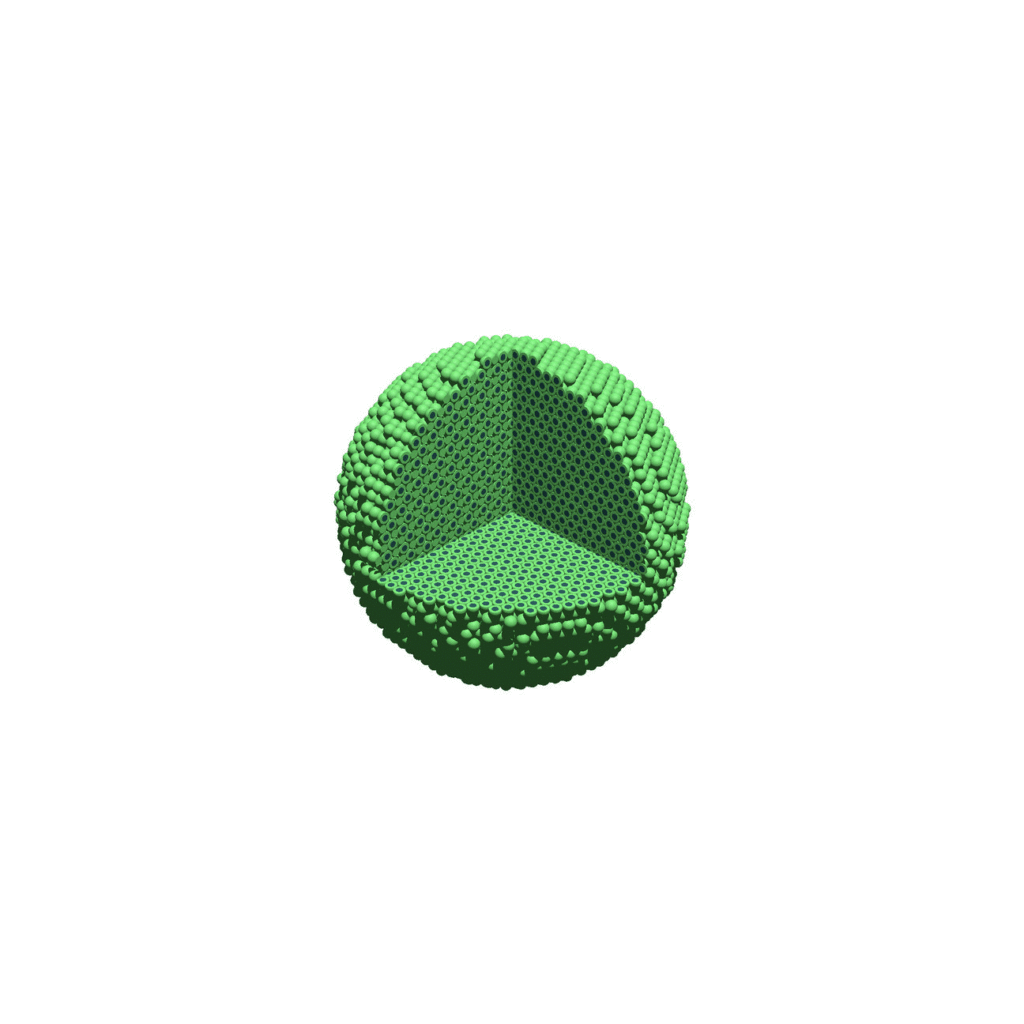Animated GIF created from raytraced still images. (You have to click the image to see the animation.) #### Creating a compressed movie with Mencoder Syntax coming later. ### Closing thoughts and future work In the future, we will probably allow more control over the clipping planes and a bit more debugging on how to handle planes that don’t pass through the origin. (First thoughts: we need to change how we use union and intersection commands in the POV-ray outputs.) We should also look at adding some transparency for the cells. I’d prefer something like rgba (red-green-blue-alpha), but POV-ray uses filters and transmission, and we want to make sure to get it right. Lastly, it would be nice to find a balance between the current very simple camera setup and better control. Thanks for reading this PhysiCell Friday tutorial! Please do give PhysiCell at try (at http://PhysiCell.org) and read the method paper at PLoS Computational Biology. Share this: Tags : ## Setting up the PhysiCell microenvironment with XML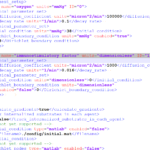As of release 1.6.0, users can define all the chemical substrates in the microenvironment with an XML configuration file. (These are stored by default in ./config/. The default parameter file is ./config/PhysiCell_settings.xml.) This should make it much easier to set up the microenvironment (previously required a lot of manual C++), as well as make it easier to change parameters and initial conditions. In release 1.7.0, users gained finer grained control on Dirichlet conditions: individual Dirichlet conditions can be enabled or disabled for each individual diffusing substrate on each individual boundary. See details below. This tutorial will show you the key techniques to use these features. (See the User_Guide for full documentation.) First, let’s create a barebones 2D project by populating the 2D template project. In a terminal shell in your root PhysiCell directory, do this: make template2D  We will use this 2D project template for the remainder of the tutorial. We assume you already have a working copy of PhysiCell installed, version 1.6.0 or later. (If not, visit the PhysiCell tutorials to find installation instructions for your operating system.) You will need version 1.7.0 or later to control Dirichlet conditions on individual boundaries. You can download the latest version of PhysiCell at: ### Microenvironment setup in the XML configuration file Next, let’s look at the parameter file. In your text editor of choice, open up ./config/PhysiCell_settings.xml, and browse down to <microenvironment_setup>: <microenvironment_setup> <variable name="oxygen" units="mmHg" ID="0"> <physical_parameter_set> <diffusion_coefficient units="micron^2/min">100000.0</diffusion_coefficient> <decay_rate units="1/min">0.1</decay_rate> </physical_parameter_set> <initial_condition units="mmHg">38.0</initial_condition> <Dirichlet_boundary_condition units="mmHg" enabled="true">38.0</Dirichlet_boundary_condition> </variable> <options> <calculate_gradients>false</calculate_gradients> <track_internalized_substrates_in_each_agent>false</track_internalized_substrates_in_each_agent> <!-- not yet supported --> <initial_condition type="matlab" enabled="false"> <filename>./config/initial.mat</filename> </initial_condition> <!-- not yet supported --> <dirichlet_nodes type="matlab" enabled="false"> <filename>./config/dirichlet.mat</filename> </dirichlet_nodes> </options> </microenvironment_setup>  Notice a few trends: • The <variable> XML element (tag) is used to define a chemical substrate in the microenvironment. The attributes say that it is named oxygen, and the units of measurement are mmHg. Notice also that the ID is 0: this unique integer identifier helps for finding and accessing the substrate within your PhysiCell project. Make sure your first substrate ID is 0, since C++ starts indexing at 0. • Within the <variable> block, we set the properties of this substrate: • <diffusion_coefficient> sets the (uniform) diffusion constant for the substrate. • <decay_rate> is the substrate’s background decay rate. • <initial_condition> is the value the substrate will be (uniformly) initialized to throughout the domain. • <Dirichlet_boundary_condition> is the value the substrate will be set to along the outer computational boundary throughout the simulation, if you set enabled=true. If enabled=false, then PhysiCell (via BioFVM) will use Neumann (zero flux) conditions for that substrate. • The <options> element helps configure other simulation behaviors: • Use <calculate_gradients> to control whether PhysiCell computes all chemical gradients at each time step. Set this to true to enable accurate gradients (e.g., for chemotaxis). • Use <track_internalized_substrates_in_each_agent> to enable or disable the PhysiCell feature of actively tracking the total amount of internalized substrates in each individual agent. Set this to true to enable the feature. • <initial_condition> is reserved for a future release where we can specify non-uniform initial conditions as an external file (e.g., a CSV or Matlab file). This is not yet supported. • <dirichlet_nodes> is reserved for a future release where we can specify Dirchlet nodes at any location in the simulation domain with an external file. This will be useful for irregular domains, but it is not yet implemented. Note that PhysiCell does not convert units. The units attributes are helpful for clarity between users and developers, to ensure that you have worked in consistent length and time units. By default, PhysiCell uses minutes for all time units, and microns for all spatial units. ### Changing an existing substrate Let’s modify the oxygen variable to do the following: • Change the diffusion coefficient to 120000 $$\mu\mathrm{m}^2 / \mathrm{min}$$ • Change the initial condition to 40 mmHg • Change the oxygen Dirichlet boundary condition to 42.7 mmHg • Enable gradient calculations If you modify the appropriate fields in the <microenvironment_setup> block, it should look like this: <microenvironment_setup> <variable name="oxygen" units="mmHg" ID="0"> <physical_parameter_set> <diffusion_coefficient units="micron^2/min">120000.0</diffusion_coefficient> <decay_rate units="1/min">0.1</decay_rate> </physical_parameter_set> <initial_condition units="mmHg">40.0</initial_condition> <Dirichlet_boundary_condition units="mmHg" enabled="true">42.7</Dirichlet_boundary_condition> </variable> <options> <calculate_gradients>true</calculate_gradients> <track_internalized_substrates_in_each_agent>false</track_internalized_substrates_in_each_agent> <!-- not yet supported --> <initial_condition type="matlab" enabled="false"> <filename>./config/initial.mat</filename> </initial_condition> <!-- not yet supported --> <dirichlet_nodes type="matlab" enabled="false"> <filename>./config/dirichlet.mat</filename> </dirichlet_nodes> </options> </microenvironment_setup>  ### Adding a new diffusing substrate Let’s add a new dimensionless substrate glucose with the following: • Diffusion coefficient is 18000 $$\mu\mathrm{m}^2 / \mathrm{min}$$ • No decay rate • The initial condition is 1 (dimensionless) • Neumann (no flux) boundary conditions To add the new variable, I suggest copying an existing variable (in this case, oxygen) and modifying to: • change the name and units throughout • increase the ID by one • write in the appropriate initial and boundary conditions If you modify the appropriate fields in the <microenvironment_setup> block, it should look like this: <microenvironment_setup> <variable name="oxygen" units="mmHg" ID="0"> <physical_parameter_set> <diffusion_coefficient units="micron^2/min">120000.0</diffusion_coefficient> <decay_rate units="1/min">0.1</decay_rate> </physical_parameter_set> <initial_condition units="mmHg">40.0</initial_condition> <Dirichlet_boundary_condition units="mmHg" enabled="true">42.7</Dirichlet_boundary_condition> </variable> <variable name="glucose" units="dimensionless" ID="1"> <physical_parameter_set> <diffusion_coefficient units="micron^2/min">18000.0</diffusion_coefficient> <decay_rate units="1/min">0.0</decay_rate> </physical_parameter_set> <initial_condition units="dimensionless">1</initial_condition> <Dirichlet_boundary_condition units="dimensionless" enabled="false">0</Dirichlet_boundary_condition> </variable> <options> <calculate_gradients>true</calculate_gradients> <track_internalized_substrates_in_each_agent>false</track_internalized_substrates_in_each_agent> <!-- not yet supported --> <initial_condition type="matlab" enabled="false"> <filename>./config/initial.mat</filename> </initial_condition> <!-- not yet supported --> <dirichlet_nodes type="matlab" enabled="false"> <filename>./config/dirichlet.mat</filename> </dirichlet_nodes> </options> </microenvironment_setup>  ### Controlling Dirichlet conditions on individual boundaries In Version 1.7.0, we introduced the ability to control the Dirichlet conditions for each individual boundary for each substrate. The examples above apply (enable) or disable the same condition on each boundary with the same boundary value. Suppose that we want to set glucose so that the Dirichlet condition is enabled on the bottom z boundary (with value 1) and the left and right x boundaries (with value 0.5) and disabled on all other boundaries. We modify the variable block by adding the optional Dirichlet_options block: <variable name="glucose" units="dimensionless" ID="1"> <physical_parameter_set> <diffusion_coefficient units="micron^2/min">18000.0</diffusion_coefficient> <decay_rate units="1/min">0.0</decay_rate> </physical_parameter_set> <initial_condition units="dimensionless">1</initial_condition> <Dirichlet_boundary_condition units="dimensionless" enabled="true">0</Dirichlet_boundary_condition> <Dirichlet_options> <boundary_value ID="xmin" enabled="true">0.5</boundary_value> <boundary_value ID="xmax" enabled="true">0.5</boundary_value> <boundary_value ID="ymin" enabled="false">0.5</boundary_value> <boundary_value ID="ymin" enabled="false">0.5</boundary_value> <boundary_value ID="zmin" enabled="true">1.0</boundary_value> <boundary_value ID="zmax" enabled="false">0.5</boundary_value> </Dirichlet_options> </variable>  Notice a few things: 1. The Dirichlet_boundary_condition element has its enabled attribute set to true 2. The Dirichlet condition is set under any individual boundary with a boundary_value element. • The ID attribute indicates which boundary is being specified. • The enabled attribute allows the individual boundary to be enabled (with value given by the element’s value) or disabled (applying a Neumann or no-flux condition for this substrate at this boundary). • Any individual boundary indicated by a boundary_value element supersedes the value given by Dirichlet_boundary_condition for this boundary. ### Closing thoughts and future work In the future, we plan to develop more of the options to allow users to set set the initial conditions externally and import them (via an external file), and to allow them to set up more complex domains by importing Dirichlet nodes. More broadly, we are working to push more model specification from raw C++ to imported XML. It is our hope that this will vastly simplify model development, facilitate creation of graphical model editing tools, and ultimately broaden the class of developers who can use and contribute to PhysiCell. Thanks for giving it a try! Share this: Tags : ## Working with PhysiCell MultiCellDS digital snapshots in MatlabPhysiCell 1.2.1 and later saves data as a specialized MultiCellDS digital snapshot, which includes chemical substrate fields, mesh information, and a readout of the cells and their phenotypes at single simulation time point. This tutorial will help you learn to use the matlab processing files included with PhysiCell. This tutorial assumes you know (1) how to work at the shell / command line of your operating system, and (2) basic plotting and other functions in Matlab. ### Key elements of a PhysiCell digital snapshot A PhysiCell digital snapshot (a customized form of the MultiCellDS digital simulation snapshot) includes the following elements saved as XML and MAT files: 1. output12345678.xml : This is the “base” output file, in MultiCellDS format. It includes key metadata such as when the file was created, the software, microenvironment information, and custom data saved at the simulation time. The Matlab files read this base file to find other related files (listed next). Example: output00003696.xml 2. initial_mesh0.mat : This is the computational mesh information for BioFVM at time 0.0. Because BioFVM and PhysiCell do not use moving meshes, we do not save this data at any subsequent time. 3. output12345678_microenvironment0.mat : This saves each biochemical substrate in the microenvironment at the computational voxels defined in the mesh (see above). Example: output00003696_microenvironment0.mat 4. output12345678_cells.mat : This saves very basic cellular information related to BioFVM, including cell positions, volumes, secretion rates, uptake rates, and secretion saturation densities. Example: output00003696_cells.mat 5. output12345678_cells_physicell.mat : This saves extra PhysiCell data for each cell agent, including volume information, cell cycle status, motility information, cell death information, basic mechanics, and any user-defined custom data. Example: output00003696_cells_physicell.mat These snapshots make extensive use of Matlab Level 4 .mat files, for fast, compact, and well-supported saving of array data. Note that even if you cannot ready MultiCellDS XML files, you can work to parse the .mat files themselves. ### The PhysiCell Matlab .m files Every PhysiCell distribution includes some matlab functions to work with PhysiCell digital simulation snapshots, stored in the matlab subdirectory. The main ones are: 1. composite_cutaway_plot.m : provides a quick, coarse 3-D cutaway plot of the discrete cells, with different colors for live (red), apoptotic (b), and necrotic (black) cells. 2. read_MultiCellDS_xml.m : reads the “base” PhysiCell snapshot and its associated matlab files. 3. set_MCDS_constants.m : creates a data structure MCDS_constants that has the same constants as PhysiCell_constants.h. This is useful for identifying cell cycle phases, etc. 4. simple_cutaway_plot.m : provides a quick, coarse 3-D cutaway plot of user-specified cells. 5. simple_plot.m : provides, a quick, coarse 3-D plot of the user-specified cells, without a cutaway or cross-sectional clipping plane. #### A note on GNU Octave Unfortunately, GNU octave does not include XML file parsing without some significant user tinkering. And one you’re done, it is approximately one order of magnitude slower than Matlab. Octave users can directly import the .mat files described above, but without the helpful metadata in the XML file. We’ll provide more information on the structure of these MAT files in a future blog post. Moreover, we plan to provide python and other tools for users without access to Matlab. ### A sample digital snapshot We provide a 3-D simulation snapshot from the final simulation time of the cancer-immune example in Ghaffarizadeh et al. (2017, in review) at: https://sourceforge.net/projects/physicell/files/Tutorials/MultiCellDS/3D_PhysiCell_matlab_sample.zip/download The corresponding SVG cross-section for that time (through = 0 μm) looks like this: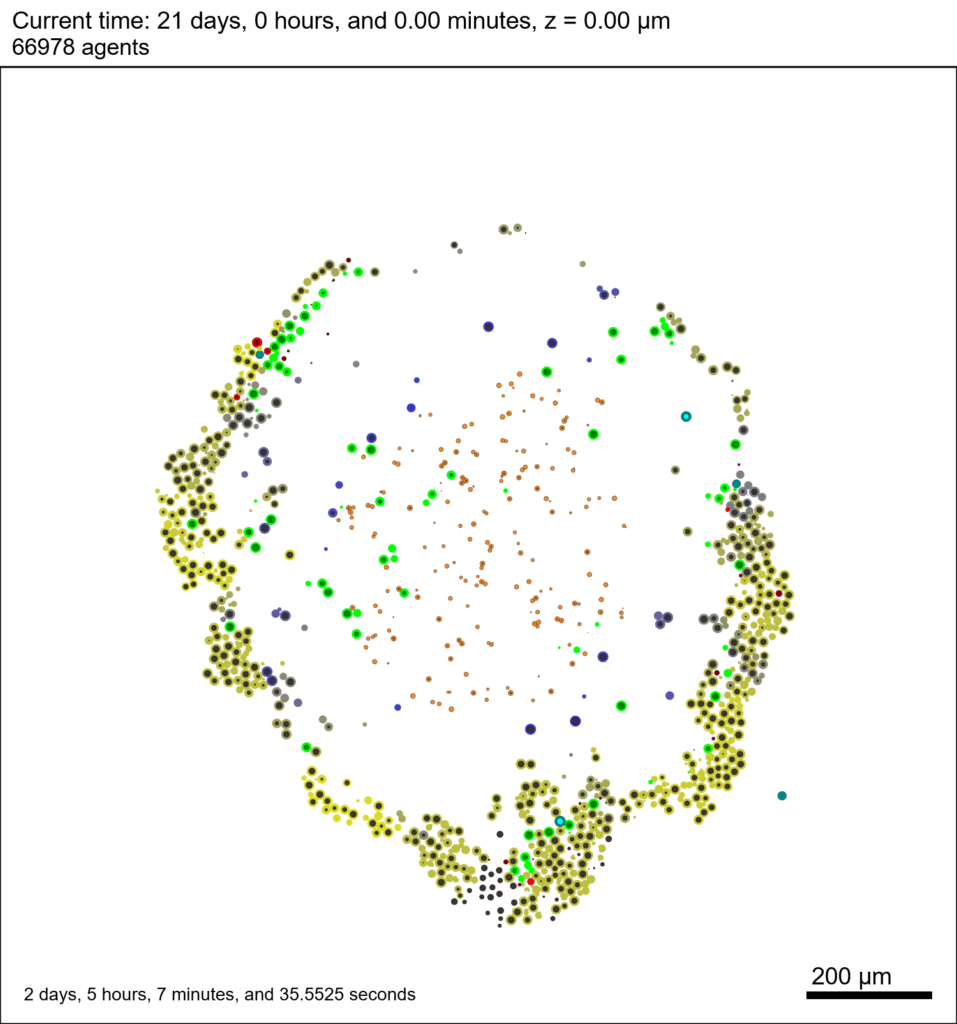Unzip the sample dataset in any directory, and make sure the matlab files above are in the same directory (or in your Matlab path). If you’re inside matlab: !unzip 3D_PhysiCell_matlab_sample.zip  ### Loading a PhysiCell MultiCellDS digital snapshot Now, load the snapshot: MCDS = read_MultiCellDS_xml( 'output00003696.xml');  This will load the mesh, substrates, and discrete cells into the MCDS data structure, and give a basic summary: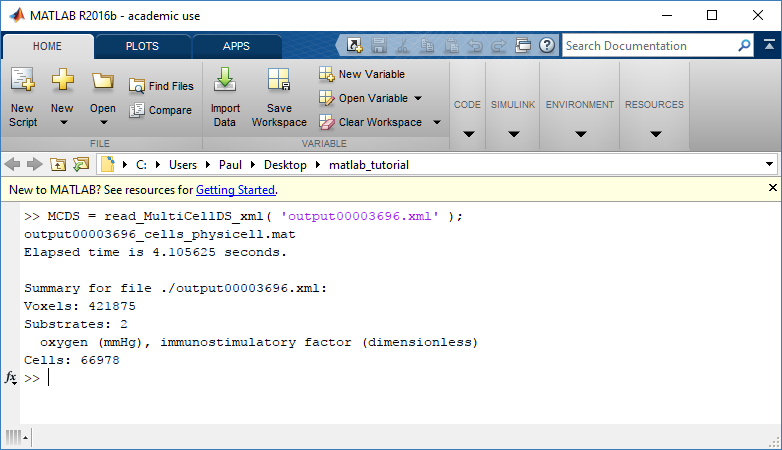Typing ‘MCDS’ and then hitting ‘tab’ (for auto-completion) shows the overall structure of MCDS, stored as metadata, mesh, continuum variables, and discrete cells: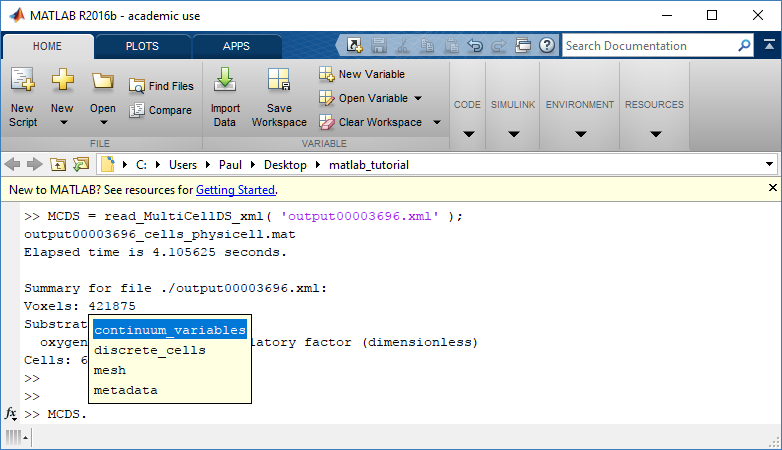To get simulation metadata, such as the current simulation time, look at MCDS.metadata.current_time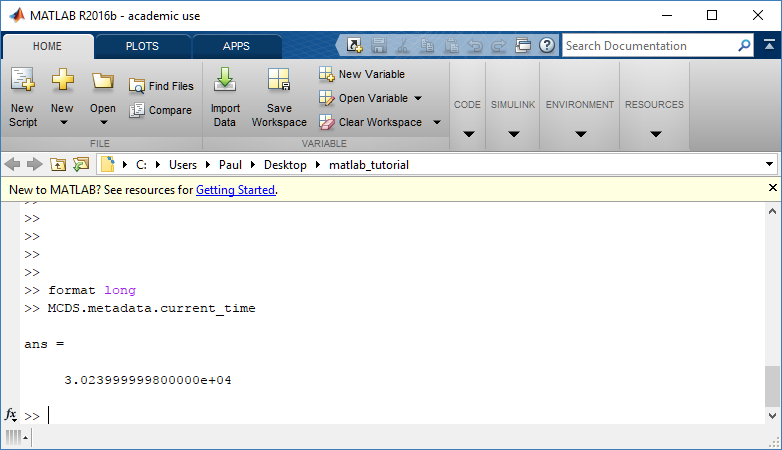Here, we see that the current simulation time is 30240 minutes, or 21 days. MCDS.metadata.current_runtime gives the elapsed walltime to up to this point: about 53 hours (1.9e5 seconds), including file I/O time to write full simulation data once per 3 simulated minutes after the start of the adaptive immune response. ### Plotting chemical substrates Let’s make an oxygen contour plot through z = 0 μm. First, we find the index corresponding to this z-value: k = find( MCDS.mesh.Z_coordinates == 0 );  Next, let’s figure out which variable is oxygen. Type “MCDS.continuum_variables.name”, which will show the array of variable names: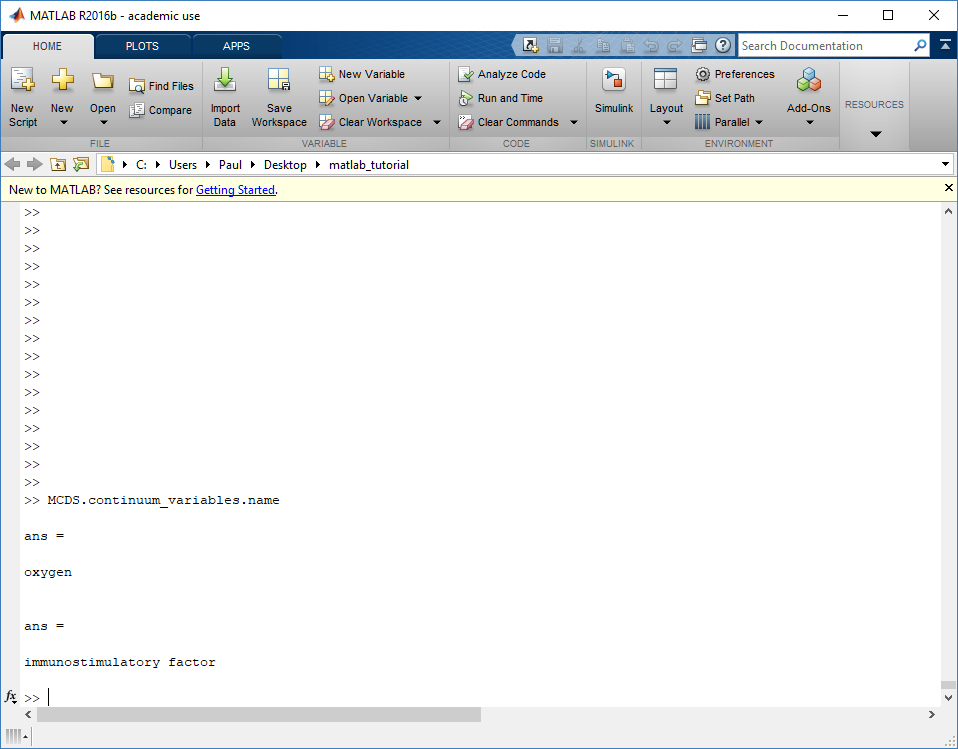Here, oxygen is the first variable, (index 1). So, to make a filled contour plot: contourf( MCDS.mesh.X(:,:,k), MCDS.mesh.Y(:,:,k), ... MCDS.continuum_variables(1).data(:,:,k) , 20 ) ;  Now, let’s set this to a correct aspect ratio (no stretching in x or y), add a colorbar, and set the axis labels, using metadata to get labels: axis image colorbar xlabel( sprintf( 'x (%s)' , MCDS.metadata.spatial_units) ); ylabel( sprintf( 'y (%s)' , MCDS.metadata.spatial_units) );  Lastly, let’s add an appropriate (time-based) title: title( sprintf('%s (%s) at t = %3.2f %s, z = %3.2f %s', MCDS.continuum_variables(1).name , ... MCDS.continuum_variables(1).units , ... MCDS.metadata.current_time , ... MCDS.metadata.time_units, ... MCDS.mesh.Z_coordinates(k), ... MCDS.metadata.spatial_units ) );  Here’s the end result: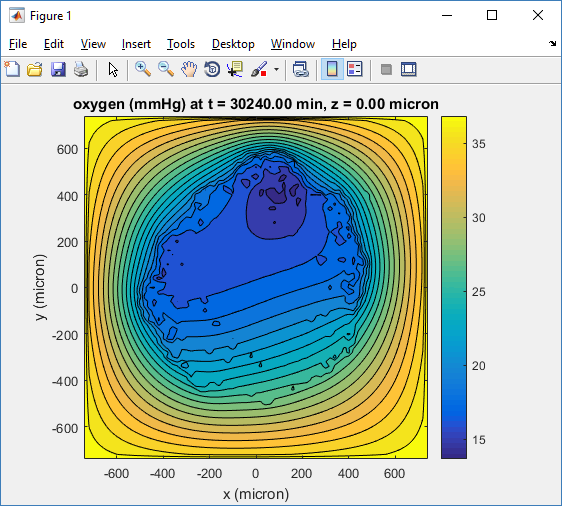We can easily export graphics, such as to PNG format: print( '-dpng' , 'output_o2.png' );  For more on plotting BioFVM data, see the tutorial at http://www.mathcancer.org/blog/saving-multicellds-data-from-biofvm/ ### Plotting cells in space #### 3-D point cloud First, let’s plot all the cells in 3D: plot3( MCDS.discrete_cells.state.position(:,1) , MCDS.discrete_cells.state.position(:,2), ... MCDS.discrete_cells.state.position(:,3) , 'bo' );  At first glance, this does not look good: some cells are far out of the simulation domain, distorting the automatic range of the plot:This does not ordinarily happen in PhysiCell (the default cell mechanics functions have checks to prevent such behavior), but this example includes a simple Hookean elastic adhesion model for immune cell attachment to tumor cells. In rare circumstances, an attached tumor cell or immune cell can apoptose on its own (due to its background apoptosis rate), without “knowing” to detach itself from the surviving cell in the pair. The remaining cell attempts to calculate its elastic velocity based upon an invalid cell position (no longer in memory), creating an artificially large velocity that “flings” it out of the simulation domain. Such cells are not simulated any further, so this is effectively equivalent to an extra apoptosis event (only 3 cells are out of the simulation domain after tens of millions of cell-cell elastic adhesion calculations). Future versions of this example will include extra checks to prevent this rare behavior. The plot can simply be fixed by changing the axis: axis( 1000*[-1 1 -1 1 -1 1] ) axis square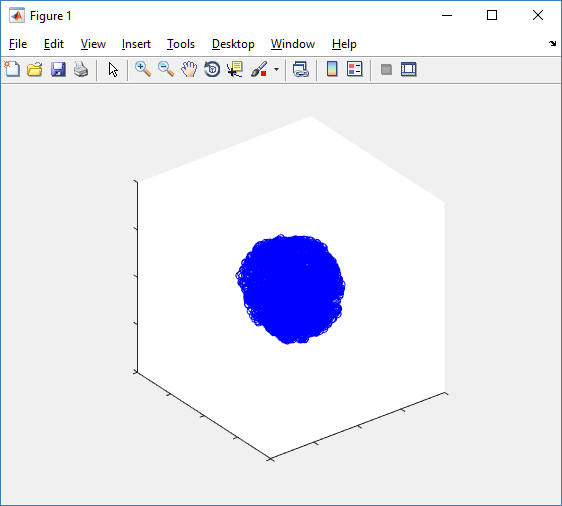Notice that this is a very difficult plot to read, and very non-interactive (laggy) to rotation and scaling operations. We can make a slightly nicer plot by searching for different cell types and plotting them with different colors: % make it easier to work with the cell positions; P = MCDS.discrete_cells.state.position; % find type 1 cells ind1 = find( MCDS.discrete_cells.metadata.type == 1 ); % better still, eliminate those out of the simulation domain ind1 = find( MCDS.discrete_cells.metadata.type == 1 & ... abs(P(:,1))' < 1000 & abs(P(:,2))' < 1000 & abs(P(:,3))' < 1000 ); % find type 0 cells ind0 = find( MCDS.discrete_cells.metadata.type == 0 & ... abs(P(:,1))' < 1000 & abs(P(:,2))' < 1000 & abs(P(:,3))' < 1000 ); %now plot them P = MCDS.discrete_cells.state.position; plot3( P(ind0,1), P(ind0,2), P(ind0,3), 'bo' ) hold on plot3( P(ind1,1), P(ind1,2), P(ind1,3), 'ro' ) hold off axis( 1000*[-1 1 -1 1 -1 1] ) axis square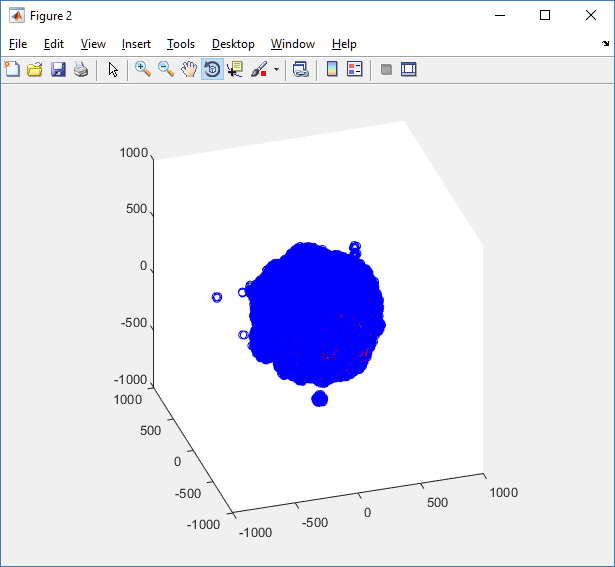However, this isn’t much better. You can use the scatter3 function to gain more control on the size and color of the plotted cells, or even make macros to plot spheres in the cell locations (with shading and lighting), but Matlab is very slow when plotting beyond 103 cells. Instead, we recommend the faster preview functions below for data exploration, and higher-quality plotting (e.g., by POV-ray) for final publication- #### Fast 3-D cell data previewers Notice that plot3 and scatter3 are painfully slow for any nontrivial number of cells. We can use a few fast previewers to quickly get a sense of the data. First, let’s plot all the dead cells, and make them red: clf simple_plot( MCDS, MCDS, MCDS.discrete_cells.dead_cells , 'r' )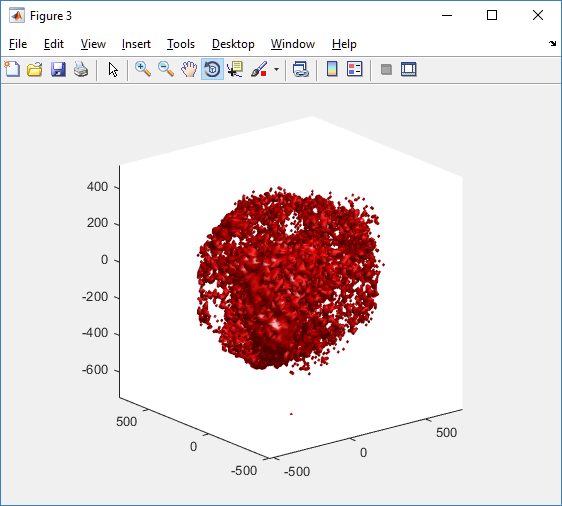This function creates a coarse-grained 3-D indicator function (0 if no cells are present; 1 if they are), and plots a 3-D level surface. It is very responsive to rotations and other operations to explore the data. You may notice the second argument is a list of indices: only these cells are plotted. This gives you a method to select cells with specific characteristics when plotting. (More on that below.) If you want to get a sense of the interior structure, use a cutaway plot: clf simple_cutaway_plot( MCDS, MCDS, MCDS.discrete_cells.dead_cells , 'r' )We also provide a fast “composite” cutaway which plots all live cells as red, apoptotic cells as blue (without the cutaway), and all necrotic cells as black: clf composite_cutaway_plot( MCDS )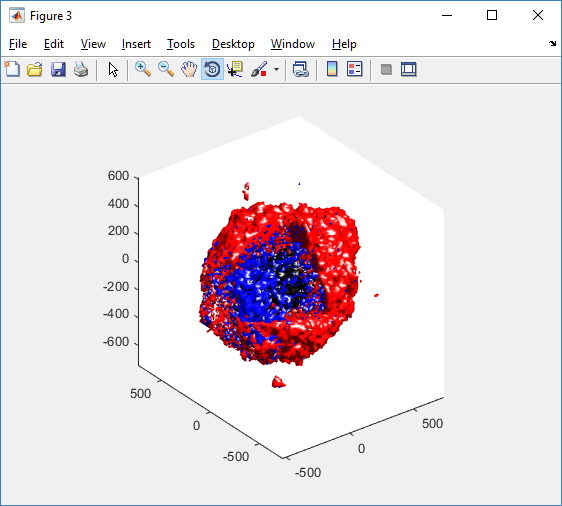Lastly, we show an improved plot that uses different colors for the immune cells, and Matlab’s “find” function to help set up the indexing: constants = set_MCDS_constants % find the type 0 necrotic cells ind0_necrotic = find( MCDS.discrete_cells.metadata.type == 0 & ... (MCDS.discrete_cells.phenotype.cycle.current_phase == constants.necrotic_swelling | ... MCDS.discrete_cells.phenotype.cycle.current_phase == constants.necrotic_lysed | ... MCDS.discrete_cells.phenotype.cycle.current_phase == constants.necrotic) ); % find the live type 0 cells ind0_live = find( MCDS.discrete_cells.metadata.type == 0 & ... (MCDS.discrete_cells.phenotype.cycle.current_phase ~= constants.necrotic_swelling & ... MCDS.discrete_cells.phenotype.cycle.current_phase ~= constants.necrotic_lysed & ... MCDS.discrete_cells.phenotype.cycle.current_phase ~= constants.necrotic & ... MCDS.discrete_cells.phenotype.cycle.current_phase ~= constants.apoptotic) ); clf % plot live tumor cells red, in cutaway view simple_cutaway_plot( MCDS, ind0_live , 'r' ); hold on % plot dead tumor cells black, in cutaway view simple_cutaway_plot( MCDS, ind0_necrotic , 'k' ) % plot all immune cells, but without cutaway (to show how they infiltrate) simple_plot( MCDS, ind1, 'g' ) hold off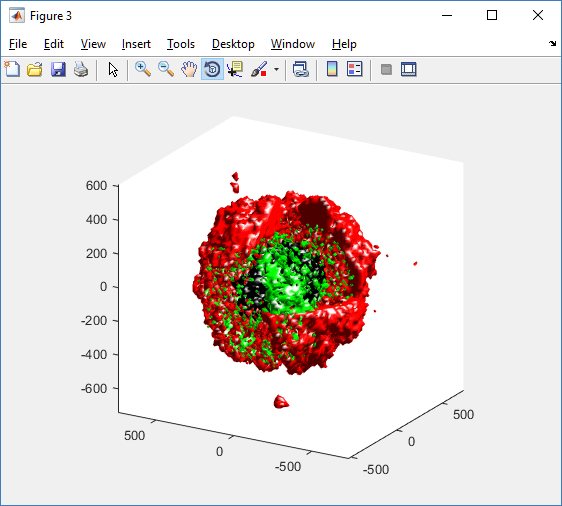### A small cautionary note on future compatibility PhysiCell 1.2.1 uses the <custom> data tag (allowed as part of the MultiCellDS specification) to encode its cell data, to allow a more compact data representation, because the current PhysiCell daft does not support such a formulation, and Matlab is painfully slow at parsing XML files larger than ~50 MB. Thus, PhysiCell snapshots are not yet fully compatible with general MultiCellDS tools, which would by default ignore custom data. In the future, we will make available converter utilities to transform “native” custom PhysiCell snapshots to MultiCellDS snapshots that encode all the cellular information in a more verbose but compatible XML format. ### Closing words and future work Because Octave is not a great option for parsing XML files (with critical MultiCellDS metadata), we plan to write similar functions to read and plot PhysiCell snapshots in Python, as an open source alternative. Moreover, our lab in the next year will focus on creating further MultiCellDS configuration, analysis, and visualization routines. We also plan to provide additional 3-D functions for plotting the discrete cells and varying color with their properties. In the longer term, we will develop open source, stand-alone analysis and visualization tools for MultiCellDS snapshots (including PhysiCell snapshots). Please stay tuned! Share this: Tags : ## Building a Cellular Automaton Model Using BioFVM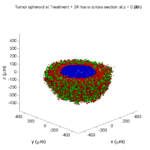Note: This is part of a series of “how-to” blog posts to help new users and developers of BioFVM. See below for guides to setting up a C++ compiler in Windows or OSX. ### What you’ll need Matlab or Octave for visualization. Matlab might be available for free at your university. Octave is open source and available from a variety of sources. ### Our modeling task We will implement a basic 3-D cellular automaton model of tumor growth in a well-mixed fluid, containing oxygen pO2 (mmHg) and a drug c (e.g., doxorubicin, μM), inspired by modeling by Alexander Anderson, Heiko Enderling, Jan PoleszczukGibin Powathil, and others. (I highly suggest seeking out the sophisticated cellular automaton models at Moffitt’s Integrated Mathematical Oncology program!) This example shows you how to extend BioFVM into a new cellular automaton model. I’ll write a similar post on how to add BioFVM into an existing cellular automaton model, which you may already have available. Tumor growth will be driven by oxygen availability. Tumor cells can be live, apoptotic (going through energy-dependent cell death, or necrotic (undergoing death from energy collapse). Drug exposure can both trigger apoptosis and inhibit cell cycling. We will model this as growth into a well-mixed fluid, with pO2 = 38 mmHg (about 5% oxygen: a physioxic value) and c = 5 μM. ### Mathematical model As a cellular automaton model, we will divide 3-D space into a regular lattice of voxels, with length, width, and height of 15 μm. (A typical breast cancer cell has radius around 9-10 μm, giving a typical volume around 3.6×103 μm3. If we make each lattice site have the volume of one cell, this gives an edge length around 15 μm.) In voxels unoccupied by cells, we approximate a well-mixed fluid with Dirichlet nodes, setting pO2 = 38 mmHg, and initially setting c = 0. Whenever a cell dies, we replace it with an empty automaton, with no Dirichlet node. Oxygen and drug follow the typical diffusion-reaction equations: $\frac{ \partial \textrm{pO}_2 }{\partial t} = D_\textrm{oxy} \nabla^2 \textrm{pO}_2 – \lambda_\textrm{oxy} \textrm{pO}_2 – \sum_{ \textrm{cells} i} U_{i,\textrm{oxy}} \textrm{pO}_2$ $\frac{ \partial c}{ \partial t } = D_c \nabla^2 c – \lambda_c c – \sum_{\textrm{cells }i} U_{i,c} c$ where each uptake rate is applied across the cell’s volume. We start the treatment by setting c = 5 μM on all Dirichlet nodes at t = 504 hours (21 days). For simplicity, we do not model drug degradation (pharmacokinetics), to approximate the in vitro conditions. In any time interval [t,tt], each live tumor cell i has a probability pi,D of attempting division, probability pi,A of apoptotic death, and probability pi,N of necrotic death. (For simplicity, we ignore motility in this version.) We relate these to the birth rate bi, apoptotic death rate di,A, and necrotic death rate di,N by the linearized equations (from Macklin et al. 2012): $\textrm{Prob} \Bigl( \textrm{cell } i \textrm{ becomes apoptotic in } [t,t+\Delta t] \Bigr) = 1 – \textrm{exp}\Bigl( -d_{i,A}(t) \Delta t\Bigr) \approx d_{i,A}\Delta t$ $\textrm{Prob} \Bigl( \textrm{cell } i \textrm{ attempts division in } [t,t+\Delta t] \Bigr) = 1 – \textrm{exp}\Bigl( -b_i(t) \Delta t\Bigr) \approx b_{i}\Delta t$ $\textrm{Prob} \Bigl( \textrm{cell } i \textrm{ becomes necrotic in } [t,t+\Delta t] \Bigr) = 1 – \textrm{exp}\Bigl( -d_{i,N}(t) \Delta t\Bigr) \approx d_{i,N}\Delta t$ Each dead cell has a mean duration Ti,D, which will vary by the type of cell death. Each dead cell automaton has a probability pi,L of lysis (rupture and removal) in any time span [t,t+Δt]. The duration TD is converted to a probability of cell lysis by $\textrm{Prob} \Bigl( \textrm{dead cell } i \textrm{ lyses in } [t,t+\Delta t] \Bigr) = 1 – \textrm{exp}\Bigl( -\frac{1}{T_{i,D}} \Delta t\Bigr) \approx \frac{ \Delta t}{T_{i,D}}$ ##### (Illustrative) parameter values We use Doxy = 105 μm2/min (Ghaffarizadeh et al. 2016), and we set Ui,oxy = 20 min-1 (to give an oxygen diffusion length scale of about 70 μm, with steeper gradients than our typical 100 μm length scale). We set λoxy = 0.01 min-1 for a 1 mm diffusion length scale in fluid. We set Dc = 300 μm2/min, and Uc = 7.2×10-3 min-1 (Dc from Weinberg et al. (2007), and Ui,c twice as large as the reference value in Weinberg et al. (2007) to get a smaller diffusion length scale of about 204 μm). We set λc = 3.6×10-5 min-1 to give a drug diffusion length scale of about 2.9 mm in fluid. We use TD = 8.6 hours for apoptotic cells, and TD = 60 days for necrotic cells (Macklin et al., 2013). However, note that necrotic and apoptotic cells lose volume quickly, so one may want to revise those time scales to match the point where a cell loses 90% of its volume. #### Functional forms for the birth and death rates We model pharmacodynamics with an area-under-the-curve (AUC) type formulation. If c(t) is the drug concentration at any cell i‘s location at time t, then let its integrated exposure Ei(t) be $E_i(t) = \int_0^t c(s) \: ds$ and we model its response with a Hill function $R_i(t) = \frac{ E_i^h(t) }{ \alpha_i^h + E_i^h(t) },$ where h is the drug’s Hill exponent for the cell line, and α is the exposure for a half-maximum effect. We model the microenvironment-dependent birth rate by: $b_i(t) = \left\{ \begin{array}{lr} b_{i,P} \left( 1 – \eta_i R_i(t) \right) & \textrm{ if } \textrm{pO}_{2,P} < \textrm{pO}_2 \\ \\ b_{i,P} \left( \frac{\textrm{pO}_{2}-\textrm{pO}_{2,N}}{\textrm{pO}_{2,P}-\textrm{pO}_{2,N}}\right) \Bigl( 1 – \eta_i R_i(t) \Bigr) & \textrm{ if } \textrm{pO}_{2,N} < \textrm{pO}_2 \le \textrm{pO}_{2,P} \\ \\ 0 & \textrm{ if } \textrm{pO}_2 \le \textrm{pO}_{2,N}\end{array} \right.$ where pO2,P is the physioxic oxygen value (38 mmHg), and pO2,N is a necrotic threshold (we use 5 mmHg), and 0 < η < 1 the drug’s birth inhibition. (A fully cytostatic drug has η = 1.) We model the microenvironment-dependent apoptosis rate by: $d_{i,A}(t) = d_{i,A}^* + \Bigl( d_{i,A}^\textrm{max} – d_{i,A}^* \Bigr) R_i(t)$ where di,Amax is the maximum apoptotic death rate. We model the microenvironment-dependent necrosis rate by: $d_{i,N}(t) = \left\{ \begin{array}{lr} 0 & \textrm{ if } \textrm{pO}_{2,N} < \textrm{pO}_{2} \\ \\ d_{i,N}^* & \textrm{ if } \textrm{pO}_{2} \le \textrm{pO}_{2,N} \end{array}\right.$ for a constant value di,N*. ##### (Illustrative) parameter values We use bi,P = 0.05 hour-1 (for a 20 hour cell cycle in physioxic conditions), di,A* = 0.01 bi,P, and di,N* = 0.04 hour-1 (so necrotic cells survive around 25 hours in low oxygen conditions). We set α = 30 μM*hour (so that cells reach half max response after 6 hours’ exposure at a maximum concentration c = 5 μM), h = 2 (for a smooth effect), η = 0.25 (so that the drug is partly cytostatic), and di,Amax = 0.1 hour^-1 (so that cells survive about 10 hours after reaching maximum response). ### Building the Cellular Automaton Model in BioFVM BioFVM already includes Basic_Agents for cell-based substrate sources and sinks. We can extend these basic agents into full-fledged automata, and then arrange them in a lattice to create a full cellular automata model. Let’s sketch that out now. #### Extending Basic_Agents to Automata The main idea here is to define an Automaton class which extends (and therefore includes) the Basic_Agent class. This will give each Automaton full access to the microenvironment defined in BioFVM, including the ability to secrete and uptake substrates. We also make sure each Automaton “knows” which microenvironment it lives in (contains a pointer pMicroenvironment), and “knows” where it lives in the cellular automaton lattice. (More on that in the following paragraphs.) So, as a schematic (just sketching out the most important members of the class): class Standard_Data; // define per-cell biological data, such as phenotype, // cell cycle status, etc.. class Custom_Data; // user-defined custom data, specific to a model. class Automaton : public Basic_Agent { private: Microenvironment* pMicroenvironment; CA_Mesh* pCA_mesh; int voxel_index; protected: public: // neighbor connectivity information std::vector<Automaton*> neighbors; std::vector<double> neighbor_weights; Standard_Data standard_data; void (*current_state_rule)( Automaton& A , double ); Automaton(); void copy_parameters( Standard_Data& SD ); void overwrite_from_automaton( Automaton& A ); void set_cellular_automaton_mesh( CA_Mesh* pMesh ); CA_Mesh* get_cellular_automaton_mesh( void ) const; void set_voxel_index( int ); int get_voxel_index( void ) const; void set_microenvironment( Microenvironment* pME ); Microenvironment* get_microenvironment( void ); // standard state changes bool attempt_division( void ); void become_apoptotic( void ); void become_necrotic( void ); void perform_lysis( void ); // things the user needs to define Custom_Data custom_data; // use this rule to add custom logic void (*custom_rule)( Automaton& A , double); };  So, the Automaton class includes everything in the Basic_Agent class, some Standard_Data (things like the cell state and phenotype, and per-cell settings), (user-defined) Custom_Data, basic cell behaviors like attempting division into an empty neighbor lattice site, and user-defined custom logic that can be applied to any automaton. To avoid lots of switch/case and if/then logic, each Automaton has a function pointer for its current activity (current_state_rule), which can be overwritten any time. Each Automaton also has a list of neighbor Automata (their memory addresses), and weights for each of these neighbors. Thus, you can distance-weight the neighbors (so that corner elements are farther away), and very generalized neighbor models are possible (e.g., all lattice sites within a certain distance). When updating a cellular automaton model, such as to kill a cell, divide it, or move it, you leave the neighbor information alone, and copy/edit the information (standard_data, custom_data, current_state_rule, custom_rule). In many ways, an Automaton is just a bucket with a cell’s information in it. Note that each Automaton also “knows” where it lives (pMicroenvironment and voxel_index), and knows what CA_Mesh it is attached to (more below). #### Connecting Automata into a Lattice An automaton by itself is lost in the world–it needs to link up into a lattice organization. Here’s where we define a CA_Mesh class, to hold the entire collection of Automata, setup functions (to match to the microenvironment), and two fundamental operations at the mesh level: copying automata (for cell division), and swapping them (for motility). We have provided two functions to accomplish these tasks, while automatically keeping the indexing and BioFVM functions correctly in sync. Here’s what it looks like: class CA_Mesh{ private: Microenvironment* pMicroenvironment; Cartesian_Mesh* pMesh; std::vector<Automaton> automata; std::vector<int> iteration_order; protected: public: CA_Mesh(); // setup to match a supplied microenvironment void setup( Microenvironment& M ); // setup to match the default microenvironment void setup( void ); int number_of_automata( void ) const; void randomize_iteration_order( void ); void swap_automata( int i, int j ); void overwrite_automaton( int source_i, int destination_i ); // return the automaton sitting in the ith lattice site Automaton& operator[]( int i ); // go through all nodes according to random shuffled order void update_automata( double dt ); };  So, the CA_Mesh has a vector of Automata (which are never themselves moved), pointers to the microenvironment and its mesh, and a vector of automata indices that gives the iteration order (so that we can sample the automata in a random order). You can easily access an automaton with operator[], and copy the data from one Automaton to another with overwrite_automaton() (e.g, for cell division), and swap two Automata’s data (e.g., for cell migration) with swap_automata(). Finally, calling update_automata(dt) iterates through all the automata according to iteration_order, calls their current_state_rules and custom_rules, and advances the automata by dt. #### Interfacing Automata with the BioFVM Microenvironment The setup function ensures that the CA_Mesh is the same size as the Microenvironment.mesh, with same indexing, and that all automata have the correct volume, and dimension of uptake/secretion rates and parameters. If you declare and set up the Microenvironment first, all this is take care of just by declaring a CA_Mesh, as it seeks out the default microenvironment and sizes itself accordingly: // declare a microenvironment Microenvironment M; // do things to set it up -- see prior tutorials // declare a Cellular_Automaton_Mesh CA_Mesh CA_model; // it's already good to go, initialized to empty automata: CA_model.display();  If you for some reason declare the CA_Mesh fist, you can set it up against the microenvironment: // declare a CA_Mesh CA_Mesh CA_model; // declare a microenvironment Microenvironment M; // do things to set it up -- see prior tutorials // initialize the CA_Mesh to match the microenvironment CA_model.setup( M ); // it's already good to go, initialized to empty automata: CA_model.display();  Because each Automaton is in the microenvironment and inherits functions from Basic_Agent, it can secrete or uptake. For example, we can use functions like this one: void set_uptake( Automaton&amp; A, std::vector<double>& uptake_rates ) { extern double BioFVM_CA_diffusion_dt; // update the uptake_rates in the standard_data A.standard_data.uptake_rates = uptake_rates; // now, transfer them to the underlying Basic_Agent *(A.uptake_rates) = A.standard_data.uptake_rates; // and make sure the internal constants are self-consistent A.set_internal_uptake_constants( BioFVM_CA_diffusion_dt ); }  A function acting on an automaton can sample the microenvironment to change parameters and state. For example: void do_nothing( Automaton& A, double dt ) { return; } void microenvironment_based_rule( Automaton& A, double dt ) { // sample the microenvironment std::vector<double> MS = (*A.get_microenvironment())( A.get_voxel_index() ); // if pO2 < 5 mmHg, set the cell to a necrotic state if( MS < 5.0 ) { A.become_necrotic(); } // if drug > 5 uM, set the birth rate to zero if( MS > 5 ) { A.standard_data.birth_rate = 0.0; } // set the custom rule to something else A.custom_rule = do_nothing; return; }  #### Implementing the mathematical model in this framework We give each tumor cell a tumor_cell_rule (using this for custom_rule): void viable_tumor_rule( Automaton& A, double dt ) { // If there's no cell here, don't bother. if( A.standard_data.state_code == BioFVM_CA_empty ) { return; } // sample the microenvironment std::vector<double> MS = (*A.get_microenvironment())( A.get_voxel_index() ); // integrate drug exposure A.standard_data.integrated_drug_exposure += ( MS*dt ); A.standard_data.drug_response_function_value = pow( A.standard_data.integrated_drug_exposure, A.standard_data.drug_hill_exponent ); double temp = pow( A.standard_data.drug_half_max_drug_exposure, A.standard_data.drug_hill_exponent ); temp += A.standard_data.drug_response_function_value; A.standard_data.drug_response_function_value /= temp; // update birth rates (which themselves update probabilities) update_birth_rate( A, MS, dt ); update_apoptotic_death_rate( A, MS, dt ); update_necrotic_death_rate( A, MS, dt ); return; }  The functional tumor birth and death rates are implemented as: void update_birth_rate( Automaton& A, std::vector<double>& MS, double dt ) { static double O2_denominator = BioFVM_CA_physioxic_O2 - BioFVM_CA_necrotic_O2; A.standard_data.birth_rate = A.standard_data.drug_response_function_value; // response A.standard_data.birth_rate *= A.standard_data.drug_max_birth_inhibition; // inhibition*response; A.standard_data.birth_rate *= -1.0; // - inhibition*response A.standard_data.birth_rate += 1.0; // 1 - inhibition*response A.standard_data.birth_rate *= viable_tumor_cell.birth_rate; // birth_rate0*(1 - inhibition*response) double temp1 = MS ; // O2 temp1 -= BioFVM_CA_necrotic_O2; temp1 /= O2_denominator; A.standard_data.birth_rate *= temp1; if( A.standard_data.birth_rate < 0 ) { A.standard_data.birth_rate = 0.0; } A.standard_data.probability_of_division = A.standard_data.birth_rate; A.standard_data.probability_of_division *= dt; // dt*birth_rate*(1 - inhibition*repsonse) // linearized probability return; } void update_apoptotic_death_rate( Automaton& A, std::vector<double>& MS, double dt ) { A.standard_data.apoptotic_death_rate = A.standard_data.drug_max_death_rate; // max_rate A.standard_data.apoptotic_death_rate -= viable_tumor_cell.apoptotic_death_rate; // max_rate - background_rate A.standard_data.apoptotic_death_rate *= A.standard_data.drug_response_function_value; // (max_rate-background_rate)*response A.standard_data.apoptotic_death_rate += viable_tumor_cell.apoptotic_death_rate; // background_rate + (max_rate-background_rate)*response A.standard_data.probability_of_apoptotic_death = A.standard_data.apoptotic_death_rate; A.standard_data.probability_of_apoptotic_death *= dt; // dt*( background_rate + (max_rate-background_rate)*response ) // linearized probability return; } void update_necrotic_death_rate( Automaton& A, std::vector<double>& MS, double dt ) { A.standard_data.necrotic_death_rate = 0.0; A.standard_data.probability_of_necrotic_death = 0.0; if( MS > BioFVM_CA_necrotic_O2 ) { return; } A.standard_data.necrotic_death_rate = perinecrotic_tumor_cell.necrotic_death_rate; A.standard_data.probability_of_necrotic_death = A.standard_data.necrotic_death_rate; A.standard_data.probability_of_necrotic_death *= dt; // dt*necrotic_death_rate return; }  And each fluid voxel (Dirichlet nodes) is implemented as the following (to turn on therapy at 21 days): void fluid_rule( Automaton& A, double dt ) { static double activation_time = 504; static double activation_dose = 5.0; static std::vector<double> activated_dirichlet( 2 , BioFVM_CA_physioxic_O2 ); static bool initialized = false; if( !initialized ) { activated_dirichlet = activation_dose; initialized = true; } if( fabs( BioFVM_CA_elapsed_time - activation_time ) < 0.01 ) { int ind = A.get_voxel_index(); if( A.get_microenvironment()->mesh.voxels[ind].is_Dirichlet ) { A.get_microenvironment()->update_dirichlet_node( ind, activated_dirichlet ); } } }  At the start of the simulation, each non-cell automaton has its custom_rule set to fluid_rule, and each tumor cell Automaton has its custom_rule set to viable_tumor_rule. Here’s how: void setup_cellular_automata_model( Microenvironment& M, CA_Mesh& CAM ) { // Fill in this environment double tumor_radius = 150; double tumor_radius_squared = tumor_radius * tumor_radius; std::vector<double> tumor_center( 3, 0.0 ); std::vector<double> dirichlet_value( 2 , 1.0 ); dirichlet_value = 38; //physioxia dirichlet_value = 0; // drug for( int i=0 ; i < M.number_of_voxels() ;i++ ) { std::vector<double> displacement( 3, 0.0 ); displacement = M.mesh.voxels[i].center; displacement -= tumor_center; double r2 = norm_squared( displacement ); if( r2 > tumor_radius_squared ) // well_mixed_fluid { M.add_dirichlet_node( i, dirichlet_value ); CAM[i].copy_parameters( well_mixed_fluid ); CAM[i].custom_rule = fluid_rule; CAM[i].current_state_rule = do_nothing; } else // tumor { CAM[i].copy_parameters( viable_tumor_cell ); CAM[i].custom_rule = viable_tumor_rule; CAM[i].current_state_rule = advance_live_state; } } }  #### Overall program loop There are two inherent time scales in this problem: cell processes like division and death (happen on the scale of hours), and transport (happens on the order of minutes). We take advantage of this by defining two step sizes: double BioFVM_CA_dt = 3; std::string BioFVM_CA_time_units = "hr"; double BioFVM_CA_save_interval = 12; double BioFVM_CA_max_time = 24*28; double BioFVM_CA_elapsed_time = 0.0; double BioFVM_CA_diffusion_dt = 0.05; std::string BioFVM_CA_transport_time_units = "min"; double BioFVM_CA_diffusion_max_time = 5.0;  Every time the simulation advances by BioFVM_CA_dt (on the order of hours), we run diffusion to quasi-steady state (for BioFVM_CA_diffusion_max_time, on the order of minutes), using time steps of size BioFVM_CA_diffusion time. We performed numerical stability and convergence analyses to determine 0.05 min works pretty well for regular lattice arrangements of cells, but you should always perform your own testing! Here’s how it all looks, in a main program loop: BioFVM_CA_elapsed_time = 0.0; double next_output_time = BioFVM_CA_elapsed_time; // next time you save data while( BioFVM_CA_elapsed_time < BioFVM_CA_max_time + 1e-10 ) { // if it's time, save the simulation if( fabs( BioFVM_CA_elapsed_time - next_output_time ) < BioFVM_CA_dt/2.0 ) { std::cout << "simulation time: " << BioFVM_CA_elapsed_time << " " << BioFVM_CA_time_units << " (" << BioFVM_CA_max_time << " " << BioFVM_CA_time_units << " max)" << std::endl; char* filename; filename = new char ; sprintf( filename, "output_%6f" , next_output_time ); save_BioFVM_cellular_automata_to_MultiCellDS_xml_pugi( filename , M , CA_model , BioFVM_CA_elapsed_time ); cell_counts( CA_model ); delete [] filename; next_output_time += BioFVM_CA_save_interval; } // do the cellular automaton step CA_model.update_automata( BioFVM_CA_dt ); BioFVM_CA_elapsed_time += BioFVM_CA_dt; // simulate biotransport to quasi-steady state double t_diffusion = 0.0; while( t_diffusion < BioFVM_CA_diffusion_max_time + 1e-10 ) { M.simulate_diffusion_decay( BioFVM_CA_diffusion_dt ); M.simulate_cell_sources_and_sinks( BioFVM_CA_diffusion_dt ); t_diffusion += BioFVM_CA_diffusion_dt; } }  ### Getting and Running the Code 1. Start a project: Create a new directory for your project (I’d recommend “BioFVM_CA_tumor”), and enter the directory. Place a copy of BioFVM (the zip file) into your directory. Unzip BioFVM, and copy BioFVM*.h, BioFVM*.cpp, and pugixml* files into that directory. 2. Download the demo source code: Download the source code for this tutorial: BioFVM_CA_Example_1, version 1.0.0 or later. Unzip its contents into your project directory. Go ahead and overwrite the Makefile. 3. Edit the makefile (if needed): Note that if you are using OSX, you’ll probably need to change from “g++” to your installed compiler. See these tutorials. 4. Test the code: Go to a command line (see previous tutorials), and test: make ./BioFVM_CA_Example_1  (If you’re on windows, run BioFVM_CA_Example_1.exe.) ### Simulation Result If you run the code to completion, you will simulate 3 weeks of in vitro growth, followed by a bolus “injection” of drug. The code will simulate one one additional week under the drug. (This should take 5-10 minutes, including full simulation saves every 12 hours.) In matlab, you can load a saved dataset and check the minimum oxygenation value like this: MCDS = read_MultiCellDS_xml( 'output_504.000000.xml' ); min(min(min( MCDS.continuum_variables(1).data )))  And then you can start visualizing like this: contourf( MCDS.mesh.X_coordinates , MCDS.mesh.Y_coordinates , ... MCDS.continuum_variables(1).data(:,:,33)' ) ; axis image; colorbar xlabel('x (\mum)' , 'fontsize' , 12 ); ylabel( 'y (\mum)' , 'fontsize', 12 ); set(gca, 'fontsize', 12 ); title('Oxygenation (mmHg) at z = 0 \mum', 'fontsize', 14 ); print('-dpng', 'Tumor_o2_3_weeks.png' ); plot_cellular_automata( MCDS , 'Tumor spheroid at 3 weeks');  #### Simulation plots Here are some plots, showing (left from right) pO2 concentration, a cross-section of the tumor (red = live cells, green = apoptotic, and blue = necrotic), and the drug concentration (after start of therapy): ##### 1 week: Oxygen- and space-limited growth are restricted to the outer boundary of the tumor spheroid. ##### 2 weeks: Oxygenation is dipped below 5 mmHg in the center, leading to necrosis. ##### 3 weeks: As the tumor grows, the hypoxic gradient increases, and the necrotic core grows. The code turns on a constant 5 micromolar dose of doxorubicin at this point ##### Treatment + 12 hours: The drug has started to penetrate the tumor, triggering apoptotic death towards the outer periphery where exposure has been greatest. ##### Treatment + 24 hours: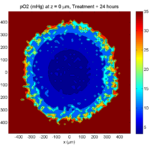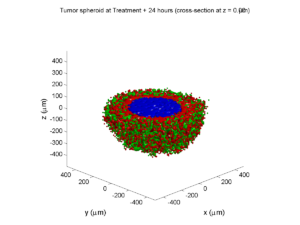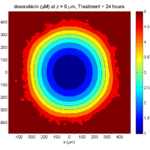The drug profile hasn’t changed much, but the interior cells have now had greater exposure to drug, and hence greater response. Now apoptosis is observed throughout the non-necrotic tumor. The tumor has decreased in volume somewhat. ##### Treatment + 36 hours: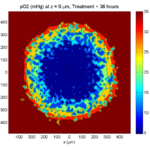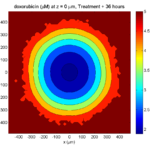The non-necrotic tumor is now substantially apoptotic. We would require some pharamcokinetic effects (e.g., drug clearance, inactivation, or removal) to avoid the inevitable, presences of a pre-existing resistant strain, or emergence of resistance. ##### Treatment + 48 hours: ##### Treatment + 60 hours: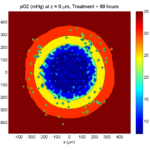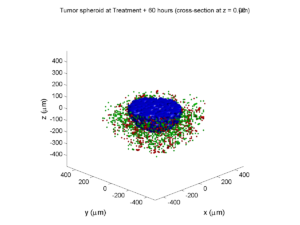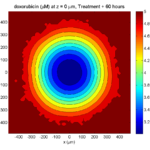The non-necrotic tumor is nearly completed eliminated, leaving a leftover core of previously-necrotic cells (which did not change state in response to the drug–they were already dead!) ### Source files You can download completed source for this example here: https://sourceforge.net/projects/biofvm/files/Tutorials/Cellular_Automaton_1/ This file will include the following: 1. BioFVM_cellular_automata.h 2. BioFVM_cellular_automata.cpp 3. BioFVM_CA_example_1.cpp 4. read_MultiCellDS_xml.m (updated) 5. plot_cellular_automata.m 6. Makefile ### What’s next I plan to update this source code with extra cell motility, and potentially more realistic parameter values. Also, I plan to more formally separate out the example from the generic cell capabilities, so that this source code can work as a bona fide cellular automaton framework. More immediately, my next tutorial will use the reverse strategy: start with an existing cellular automaton model, and integrate BioFVM capabilities. Return to News • Return to MathCancer Share this: ## Saving MultiCellDS data from BioFVM Note: This is part of a series of “how-to” blog posts to help new users and developers of BioFVM ### Introduction A major initiative for my lab has been MultiCellDS: a standard for multicellular data. The project aims to create model-neutral representations of simulation data (for both discrete and continuum models), which can also work for segmented experimental and clinical data. A single-time output is called a digital snapshot. An interdisciplinary, multi-institutional review panel has been hard at work to nail down the draft standard. A BioFVM MultiCellDS digital snapshot includes program and user metadata (more information to be included in a forthcoming publication), an output of the microenvironment, and any cells that are secreting or uptaking substrates. As of Version 1.1.0, BioFVM supports output saved to MultiCellDS XML files. Each download also includes a matlab function for importing MultiCellDS snapshots saved by BioFVM programs. This tutorial will get you going. BioFVM (finite volume method for biological problems) is an open source code for solving 3-D diffusion of 1 or more substrates. It was recently published as open access in Bioinformatics here: http://dx.doi.org/10.1093/bioinformatics/btv730 The project website is at http://BioFVM.MathCancer.org, and downloads are at http://BioFVM.sf.net. ### Working with MultiCellDS in BioFVM programs We include a MultiCellDS_test.cpp file in the examples directory of every BioFVM download (Version 1.1.0 or later). Create a new project directory, copy the following files to it: 1. BioFVM*.cpp and BioFVM*.h (from the main BioFVM directory) 2. pugixml.* (from the main BioFVM directory) 3. Makefile and MultiCellDS_test.cpp (from the examples directory) Open the MultiCellDS_test.cpp file to see the syntax as you read the rest of this post. See earlier tutorials (below) if you have troubles with this. #### Setting metadata values There are few key bits of metadata. First, the program used for the simulation (all these fields are optional): // the program name, version, and project website: BioFVM_metadata.program.program_name = "BioFVM MultiCellDS Test"; BioFVM_metadata.program.program_version = "1.0"; BioFVM_metadata.program.program_URL = "http://BioFVM.MathCancer.org"; // who created the program (if known) BioFVM_metadata.program.creator.surname = "Macklin"; BioFVM_metadata.program.creator.given_names = "Paul"; BioFVM_metadata.program.creator.email = "Paul.Macklin@usc.edu"; BioFVM_metadata.program.creator.URL = "http://BioFVM.MathCancer.org"; BioFVM_metadata.program.creator.organization = "University of Southern California"; BioFVM_metadata.program.creator.department = "Center for Applied Molecular Medicine"; BioFVM_metadata.program.creator.ORCID = "0000-0002-9925-0151"; // (generally peer-reviewed) citation information for the program BioFVM_metadata.program.citation.DOI = "10.1093/bioinformatics/btv730"; BioFVM_metadata.program.citation.PMID = "26656933"; BioFVM_metadata.program.citation.PMCID = "PMC1234567"; BioFVM_metadata.program.citation.text = "A. Ghaffarizadeh, S.H. Friedman, and P. Macklin, BioFVM: an efficient parallelized diffusive transport solver for 3-D biological simulations, Bioinformatics, 2015. DOI: 10.1093/bioinformatics/btv730."; BioFVM_metadata.program.citation.notes = "notes here"; BioFVM_metadata.program.citation.URL = "http://dx.doi.org/10.1093/bioinformatics/btv730"; // user information: who ran the program BioFVM_metadata.program.user.surname = "Kirk"; BioFVM_metadata.program.user.given_names = "James T."; BioFVM_metadata.program.user.email = "Jimmy.Kirk@starfleet.mil"; BioFVM_metadata.program.user.organization = "Starfleet"; BioFVM_metadata.program.user.department = "U.S.S. Enterprise (NCC 1701)"; BioFVM_metadata.program.user.ORCID = "0000-0000-0000-0000"; // And finally, data citation information (the publication where this simulation snapshot appeared) BioFVM_metadata.data_citation.DOI = "10.1093/bioinformatics/btv730"; BioFVM_metadata.data_citation.PMID = "12345678"; BioFVM_metadata.data_citation.PMCID = "PMC1234567"; BioFVM_metadata.data_citation.text = "A. Ghaffarizadeh, S.H. Friedman, and P. Macklin, BioFVM: an efficient parallelized diffusive transport solver for 3-D biological simulations, Bioinformatics, 2015. DOI: 10.1093/bioinformatics/btv730."; BioFVM_metadata.data_citation.notes = "notes here"; BioFVM_metadata.data_citation.URL = "http://dx.doi.org/10.1093/bioinformatics/btv730";  You can sync the metadata current time, program runtime (wall time), and dimensional units using the following command. (This command is automatically run whenever you use the save command below.) BioFVM_metadata.sync_to_microenvironment( M );  You can display a basic summary of the metadata via: BioFVM_metadata.display_information( std::cout );  #### Setting options By default (to save time and disk space), BioFVM saves the mesh as a Level 3 matlab file, whose location is embedded into the MultiCellDS XML file. You can disable this feature and revert to full XML (e.g., for human-readable cross-model reporting) via: set_save_biofvm_mesh_as_matlab( false );  Similarly, BioFVM defaults to saving the values of the substrates in a compact Level 3 matlab file. You can override this with: set_save_biofvm_data_as_matlab( false );  BioFVM by default saves the cell-centered sources and sinks. These take a lot of time to parse because they require very hierarchical data structures. You can disable saving the cells (basic_agents) via: set_save_biofvm_cell_data( false );  Lastly, when you do save the cells, we default to a customized, minimal matlab format. You can revert to a more standard (but much larger) XML format with: set_save_biofvm_cell_data_as_custom_matlab( false )  #### Saving a file Saving the data is very straightforward: save_BioFVM_to_MultiCellDS_xml_pugi( "sample" , M , current_simulation_time );  Your data will be saved in sample.xml. (Depending upon your options, it may generate several .mat files beginning with “sample”.) If you’d like the filename to depend upon the simulation time, use something more like this: double current_simulation_time = 10.347; char filename_base ; sprintf( &filename_base , "sample_%f", current_simulation_time ); save_BioFVM_to_MultiCellDS_xml_pugi( filename_base , M, current_simulation_time );  Your data will be saved in sample_10.347000.xml. (Depending upon your options, it may generate several .mat files beginning with “sample_10.347000”.) #### Compiling and running the program: Edit the Makefile as below: PROGRAM_NAME := MCDS_test all:$(BioFVM_OBJECTS) $(pugixml_OBJECTS) MultiCellDS_test.cpp$(COMPILE_COMMAND) -o $(PROGRAM_NAME)$(BioFVM_OBJECTS) \$(pugixml_OBJECTS) MultiCellDS_test.cpp


If you’re running OSX, you’ll probably need to update the compiler from “g++”. See these tutorials.

Then, at the command prompt:

make
./MCDS_test


On Windows, you’ll need to run without the ./:

make
MCDS_test


### Working with MultiCellDS data in Matlab

MCDS = read_MultiCellDS_xml( 'sample.xml' );


This should take around 30 seconds for larger data files (500,000 to 1,000,000 voxels with a few substrates, and around 250,000 cells). The long execution time is primarily because Matlab is ghastly inefficient at loops over hierarchical data structures. Increasing to 1,000,000 cells requires around 80-90 seconds to parse in matlab.

#### Plotting data in Matlab

##### Plotting the 3-D substrate data

First, let’s do some basic contour and surface plotting:

mid_index = round( length(MCDS.mesh.Z_coordinates)/2 );

contourf( MCDS.mesh.X(:,:,mid_index), ...
MCDS.mesh.Y(:,:,mid_index), ...
MCDS.continuum_variables(2).data(:,:,mid_index) , 20 ) ;
axis image
colorbar
xlabel( sprintf( 'x (%s)' , MCDS.metadata.spatial_units) );
ylabel( sprintf( 'y (%s)' , MCDS.metadata.spatial_units) );
title( sprintf('%s (%s) at t = %f %s, z = %f %s', MCDS.continuum_variables(2).name , ...
MCDS.continuum_variables(2).units , ...
MCDS.mesh.Z_coordinates(mid_index), ...


OR

contourf( MCDS.mesh.X_coordinates , MCDS.mesh.Y_coordinates, ...
MCDS.continuum_variables(2).data(:,:,mid_index) , 20 ) ;
axis image
colorbar
xlabel( sprintf( 'x (%s)' , MCDS.metadata.spatial_units) );
ylabel( sprintf( 'y (%s)' , MCDS.metadata.spatial_units) );
title( sprintf('%s (%s) at t = %f %s, z = %f %s', ...
MCDS.continuum_variables(2).name , ...
MCDS.continuum_variables(2).units , ...
MCDS.mesh.Z_coordinates(mid_index), ...


Here’s a surface plot:

surf( MCDS.mesh.X_coordinates , MCDS.mesh.Y_coordinates, ...
MCDS.continuum_variables(1).data(:,:,mid_index) ) ;
colorbar
axis tight
xlabel( sprintf( 'x (%s)' , MCDS.metadata.spatial_units) );
ylabel( sprintf( 'y (%s)' , MCDS.metadata.spatial_units) );
zlabel( sprintf( '%s (%s)', MCDS.continuum_variables(1).name, ...
MCDS.continuum_variables(1).units ) );
title( sprintf('%s (%s) at t = %f %s, z = %f %s', MCDS.continuum_variables(1).name , ...
MCDS.continuum_variables(1).units , ...
MCDS.mesh.Z_coordinates(mid_index), ...


Finally, here are some more advanced plots. The first is an “exploded” stack of contour plots:

clf
contourslice( MCDS.mesh.X , MCDS.mesh.Y, MCDS.mesh.Z , ...
MCDS.continuum_variables(2).data , [],[], ...
MCDS.mesh.Z_coordinates(1:15:length(MCDS.mesh.Z_coordinates)),20);
view([-45 10]);
axis tight;
xlabel( sprintf( 'x (%s)' , MCDS.metadata.spatial_units) );
ylabel( sprintf( 'y (%s)' , MCDS.metadata.spatial_units) );
zlabel( sprintf( 'z (%s)' , MCDS.metadata.spatial_units) );
title( sprintf('%s (%s) at t = %f %s', ...
MCDS.continuum_variables(2).name , ...
MCDS.continuum_variables(2).units , ...


Next, we show how to use isosurfaces with transparency

clf
patch( isosurface( MCDS.mesh.X , MCDS.mesh.Y, MCDS.mesh.Z, ...
MCDS.continuum_variables(1).data, 1000 ), 'edgecolor', ...
'none', 'facecolor', 'r' , 'facealpha' , 1 );
hold on
patch( isosurface( MCDS.mesh.X , MCDS.mesh.Y, MCDS.mesh.Z, ...
MCDS.continuum_variables(1).data, 5000 ), 'edgecolor', ...
'none', 'facecolor', 'b' , 'facealpha' , 0.7 );
patch( isosurface( MCDS.mesh.X , MCDS.mesh.Y, MCDS.mesh.Z, ...
MCDS.continuum_variables(1).data, 10000 ), 'edgecolor', ...
'none', 'facecolor', 'g' , 'facealpha' , 0.5 );
hold off
camlight
view(3)
axis image
axis tightcamlight lighting gouraud
xlabel( sprintf( 'x (%s)' , MCDS.metadata.spatial_units) );
ylabel( sprintf( 'y (%s)' , MCDS.metadata.spatial_units) );
zlabel( sprintf( 'z (%s)' , MCDS.metadata.spatial_units) );
title( sprintf('%s (%s) at t = %f %s', ...
MCDS.continuum_variables(1).name , ...
MCDS.continuum_variables(1).units , ...


You can get more 3-D volumetric visualization ideas at Matlab’s website. This visualization post at MIT also has some great tips.

##### Plotting the cells

Here is a basic 3-D plot for the cells:

plot3( MCDS.discrete_cells.state.position(:,1) , ...
MCDS.discrete_cells.state.position(:,2) , ...
MCDS.discrete_cells.state.position(:,3) , 'bo' );
view(3)
axis tight
xlabel( sprintf( 'x (%s)' , MCDS.metadata.spatial_units) );
ylabel( sprintf( 'y (%s)' , MCDS.metadata.spatial_units) );
zlabel( sprintf( 'z (%s)' , MCDS.metadata.spatial_units) );
title( sprintf('Cells at t = %f %s', MCDS.metadata.current_time, ...


plot3 is more efficient than scatter3, but scatter3 will give more coloring options. Here is the syntax:

scatter3( MCDS.discrete_cells.state.position(:,1), ...
MCDS.discrete_cells.state.position(:,2), ...
MCDS.discrete_cells.state.position(:,3) , 'bo' );
view(3)
axis tight
xlabel( sprintf( 'x (%s)' , MCDS.metadata.spatial_units) );
ylabel( sprintf( 'y (%s)' , MCDS.metadata.spatial_units) );
zlabel( sprintf( 'z (%s)' , MCDS.metadata.spatial_units) );
title( sprintf('Cells at t = %f %s', MCDS.metadata.current_time, ...


Jan Poleszczuk gives some great insights on plotting many cells in 3D at his blog. I’d recommend checking out his post on visualizing a cellular automaton model. At some point, I’ll update this post with prettier plotting based on his methods.

### What’s next

Future releases of BioFVM will support reading MultiCellDS snapshots (for model initialization).

Matlab is pretty slow at parsing and visualizing large amounts of data. We also plan to include resources for accessing MultiCellDS data in VTK / Paraview and Python.

## Paul Macklin calls for common standards in cancer modeling

At a recent NCI-organized mini-symposium on big data in cancer, Paul Macklin called for new data standards in Multicellular data in simulations, experiments, and clinical science. USC featured the talk (abstract here) and the work at news.usc.edu.

Read the article: http://news.usc.edu/59091/usc-researcher-calls-for-common-standards-in-cancer-modeling/ (Feb. 21, 2014)

## Paul Macklin interviewed at 2013 PSOC Annual Meeting

Paul Macklin gave a plenary talk at the 2013 NIH Physical Sciences in Oncology Annual Meeting. After the talk, he gave an interview to the Pauline Davies at the NIH on the need for data standards and model compatibility in computational and mathematical modeling of cancer. Of particular interest:

Pauline Davies: How would you ever get this standardization? Who would be responsible for saying we want it all reported in this particular way?

Paul Macklin: That’s a good question. It’s a bit of the chicken and the egg problem. Who’s going to come and give you data in your standard if you don’t have a standard? How do you plan a standard without any data? And so it’s a bit interesting. I just think someone needs to step forward and show leadership and try to get a small working group together, and at the end of the day, perfect is the enemy of the good. I think you start small and give it a go, and you add more to your standard as you need it. So maybe version one is, let’s say, how quickly the cells divide, how often they do it, how quickly they die, and what their oxygen level is, and maybe their positions. And that can be version one of this standard and a few of us try it out and see what we can do. I think it really comes down to a starting group of people and a simple starting point, and you grow it as you need it.

Shortly after, the MultiCellDS project was born (using just this strategy above!), with the generous assistance of the Breast Cancer Research Foundation.

Read / Listen to the interview: http://physics.cancer.gov/report/2013report/PaulMacklin.aspx (2013)